Скачать презентацию Chapter 13 More on sorting and searching

fff39f4bff1ffec96d66e0b814f2b165.ppt

• Количество слайдов: 83Chapter 13 » More on sorting and searching » The slides for this chapter are based on slides made by Professor Jeff Rosenschein, Computer-Science Department, The Hebrew University, Jerusalem, Israel.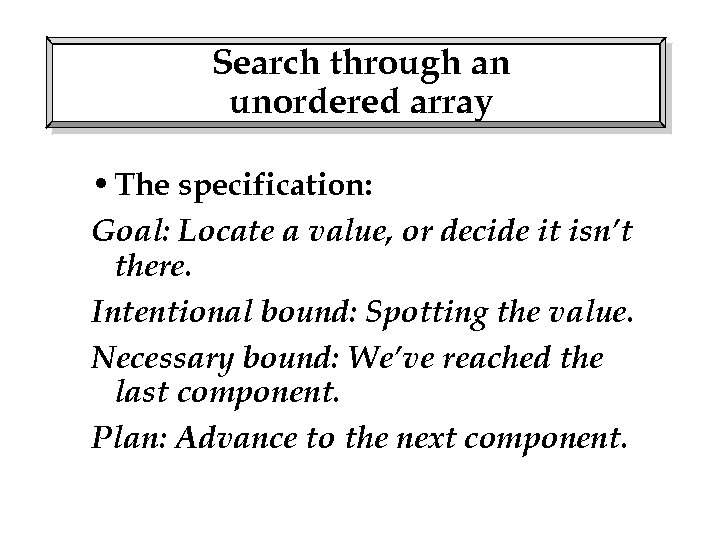Search through an unordered array • The specification: Goal: Locate a value, or decide it isn’t there. Intentional bound: Spotting the value. Necessary bound: We’ve reached the last component. Plan: Advance to the next component.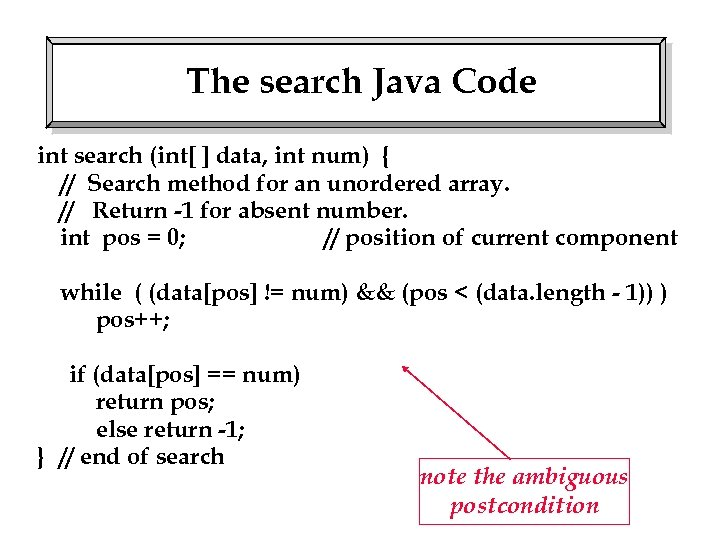The search Java Code int search (int[ ] data, int num) { // Search method for an unordered array. // Return -1 for absent number. int pos = 0; // position of current component while ( (data[pos] != num) && (pos < (data. length - 1)) ) pos++; if (data[pos] == num) return pos; else return -1; } // end of search note the ambiguous postcondition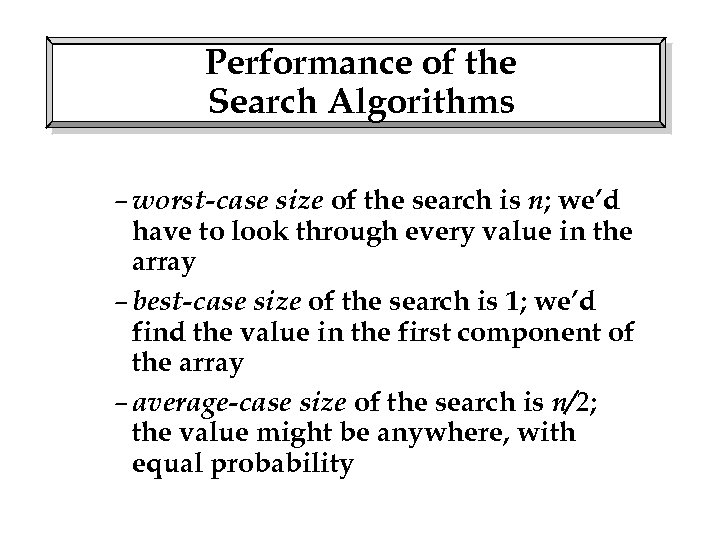Performance of the Search Algorithms – worst-case size of the search is n; we’d have to look through every value in the array – best-case size of the search is 1; we’d find the value in the first component of the array – average-case size of the search is n/2; the value might be anywhere, with equal probability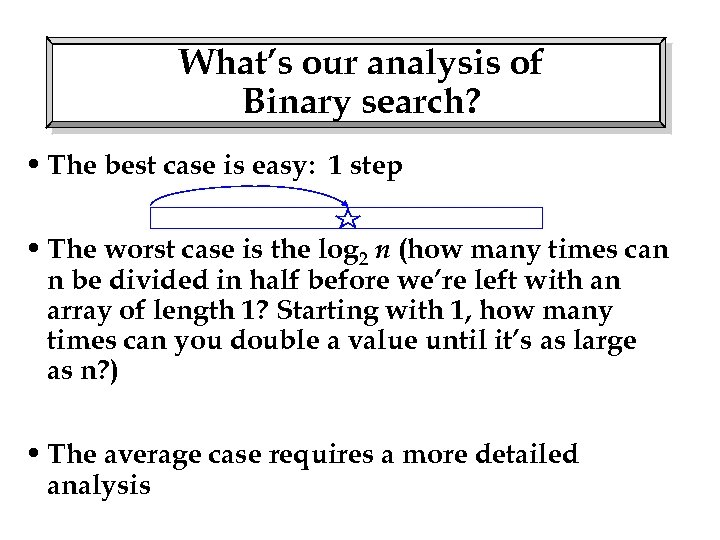What’s our analysis of Binary search? • The best case is easy: 1 step • The worst case is the log 2 n (how many times can n be divided in half before we’re left with an array of length 1? Starting with 1, how many times can you double a value until it’s as large as n? ) • The average case requires a more detailed analysis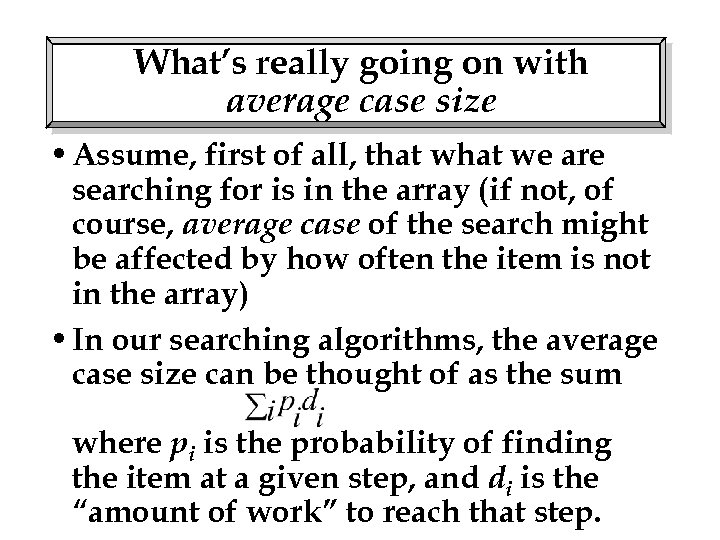What’s really going on with average case size • Assume, first of all, that we are searching for is in the array (if not, of course, average case of the search might be affected by how often the item is not in the array) • In our searching algorithms, the average case size can be thought of as the sum where pi is the probability of finding the item at a given step, and di is the “amount of work” to reach that step.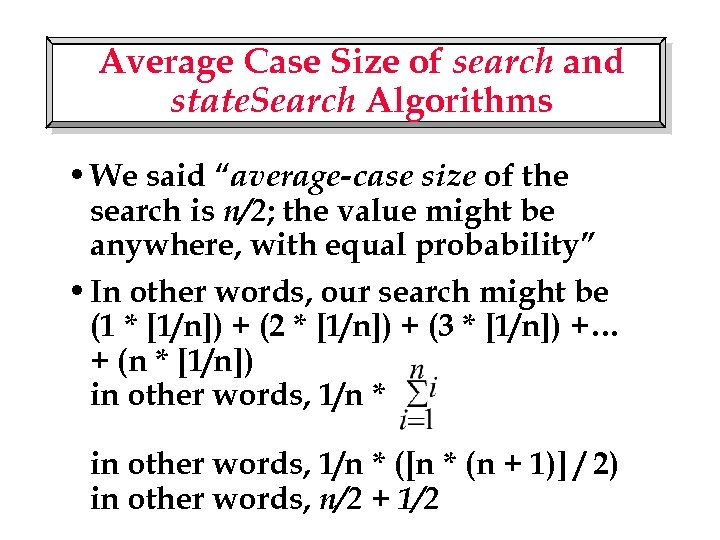Average Case Size of search and state. Search Algorithms • We said “average-case size of the search is n/2; the value might be anywhere, with equal probability” • In other words, our search might be (1 * [1/n]) + (2 * [1/n]) + (3 * [1/n]) +… + (n * [1/n]) in other words, 1/n * ([n * (n + 1)] / 2) in other words, n/2 + 1/2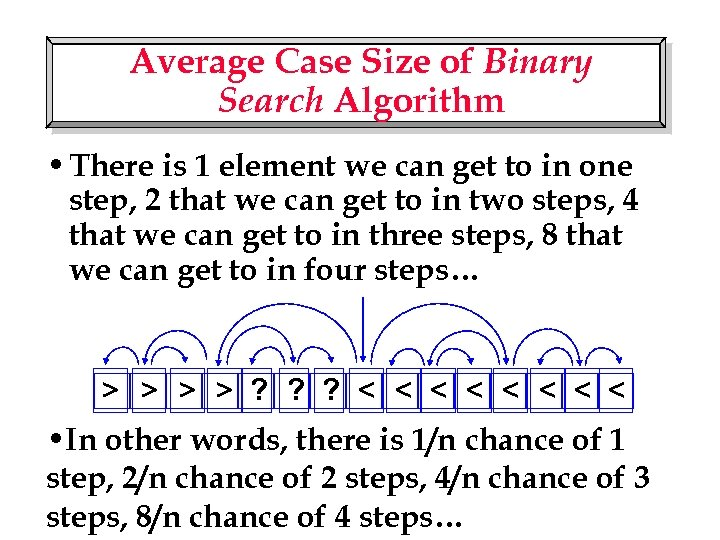Average Case Size of Binary Search Algorithm • There is 1 element we can get to in one step, 2 that we can get to in two steps, 4 that we can get to in three steps, 8 that we can get to in four steps… > > ? ? ? < < < < • In other words, there is 1/n chance of 1 step, 2/n chance of 2 steps, 4/n chance of 3 steps, 8/n chance of 4 steps…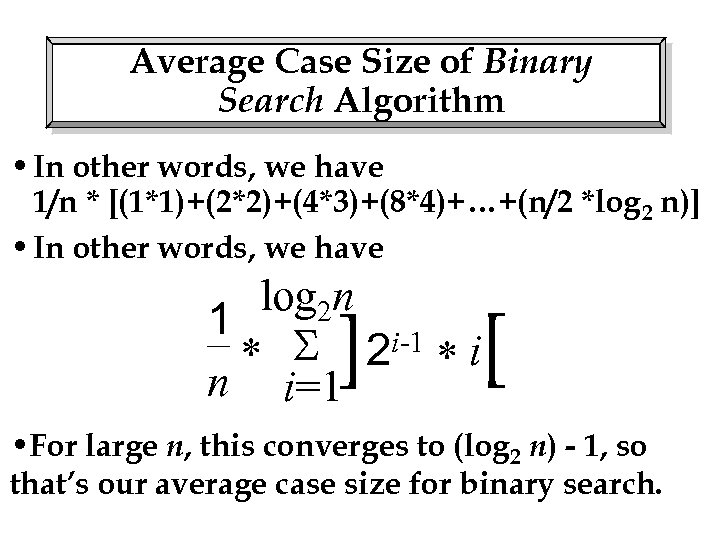Average Case Size of Binary Search Algorithm • In other words, we have 1/n * [(1*1)+(2*2)+(4*3)+(8*4)+…+(n/2 *log 2 n)] • In other words, we have log 2 n 1 * 2 i-1 * i n i=1 ] [ • For large n, this converges to (log 2 n) - 1, so that’s our average case size for binary search.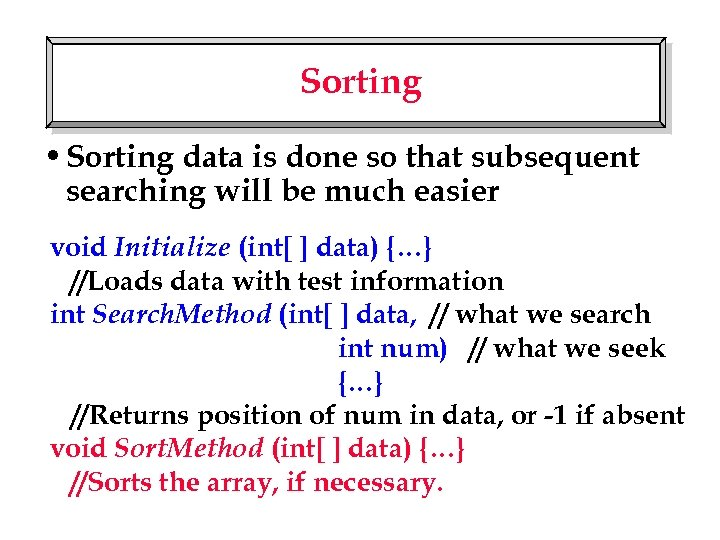Sorting • Sorting data is done so that subsequent searching will be much easier void Initialize (int[ ] data) {…} //Loads data with test information int Search. Method (int[ ] data, // what we search int num) // what we seek {…} //Returns position of num in data, or -1 if absent void Sort. Method (int[ ] data) {…} //Sorts the array, if necessary.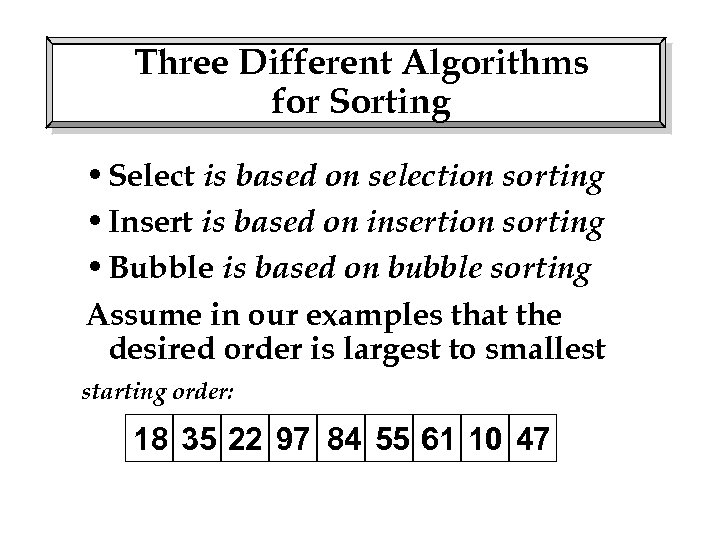Three Different Algorithms for Sorting • Select is based on selection sorting • Insert is based on insertion sorting • Bubble is based on bubble sorting Assume in our examples that the desired order is largest to smallest starting order: 18 35 22 97 84 55 61 10 47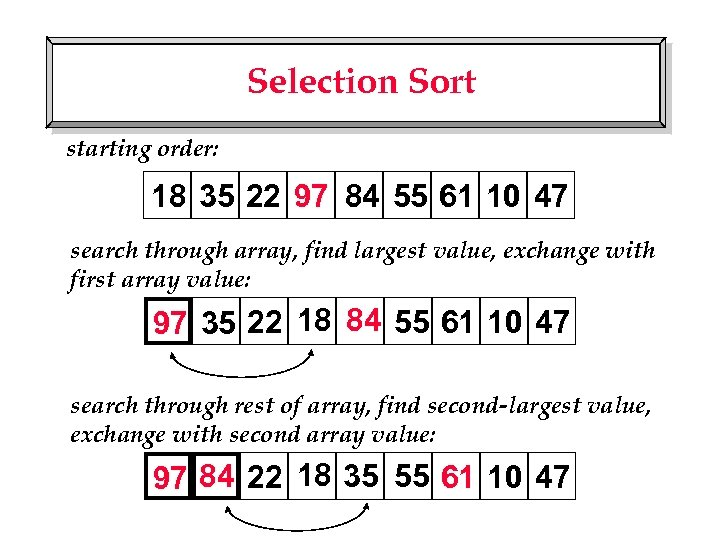Selection Sort starting order: 18 35 22 97 84 55 61 10 47 search through array, find largest value, exchange with first array value: 97 35 22 18 84 55 61 10 47 search through rest of array, find second-largest value, exchange with second array value: 97 84 22 18 35 55 61 10 47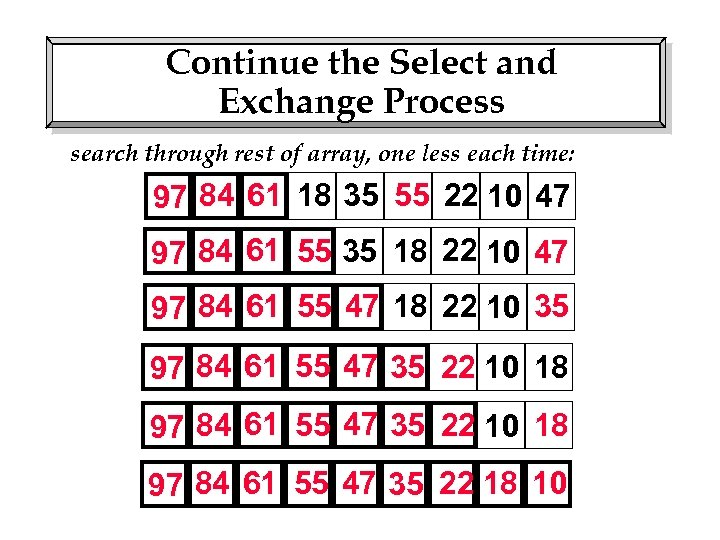Continue the Select and Exchange Process search through rest of array, one less each time: 97 84 61 18 35 55 22 10 47 97 84 61 55 35 18 22 10 47 97 84 61 55 47 18 22 10 35 97 84 61 55 47 35 22 10 18 97 84 61 55 47 35 22 18 10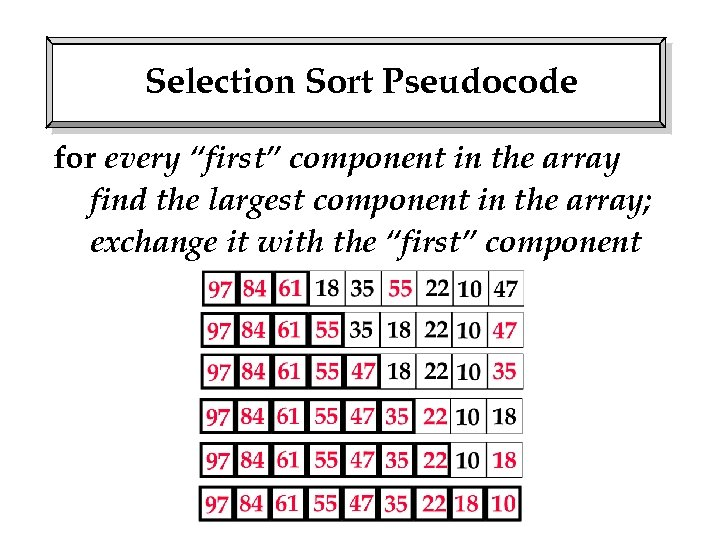Selection Sort Pseudocode for every “first” component in the array find the largest component in the array; exchange it with the “first” component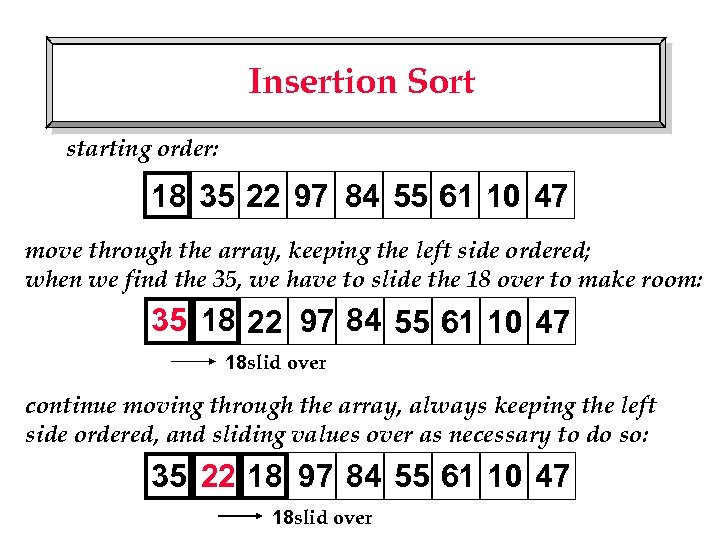Insertion Sort starting order: 18 35 22 97 84 55 61 10 47 move through the array, keeping the left side ordered; when we find the 35, we have to slide the 18 over to make room: 35 18 22 97 84 55 61 10 47 18 slid over continue moving through the array, always keeping the left side ordered, and sliding values over as necessary to do so: 35 22 18 97 84 55 61 10 47 18 slid over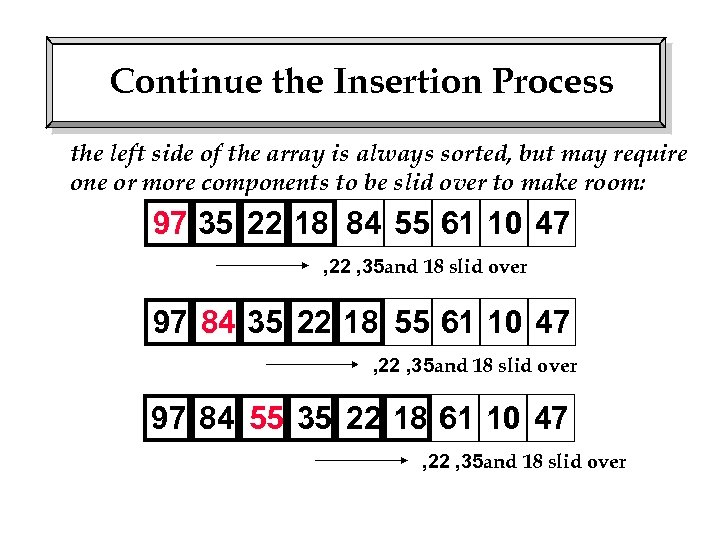Continue the Insertion Process the left side of the array is always sorted, but may require one or more components to be slid over to make room: 97 35 22 18 84 55 61 10 47 , 22 , 35 and 18 slid over 97 84 35 22 18 55 61 10 47 , 22 , 35 and 18 slid over 97 84 55 35 22 18 61 10 47 , 22 , 35 and 18 slid over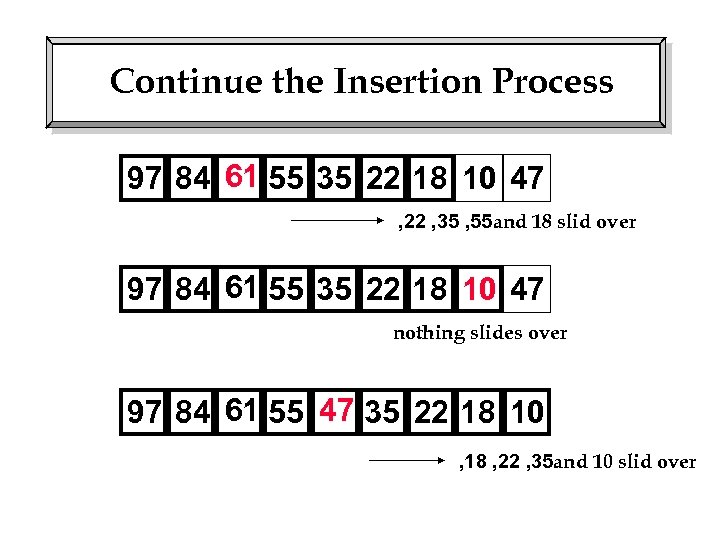Continue the Insertion Process 97 84 61 55 35 22 18 10 47 , 22 , 35 , 55 and 18 slid over 97 84 61 55 35 22 18 10 47 nothing slides over 97 84 61 55 47 35 22 18 10 , 18 , 22 , 35 and 10 slid over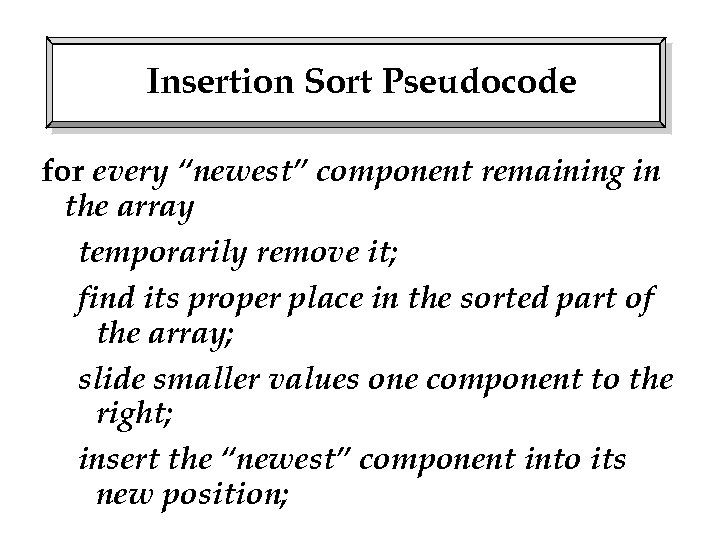Insertion Sort Pseudocode for every “newest” component remaining in the array temporarily remove it; find its proper place in the sorted part of the array; slide smaller values one component to the right; insert the “newest” component into its new position;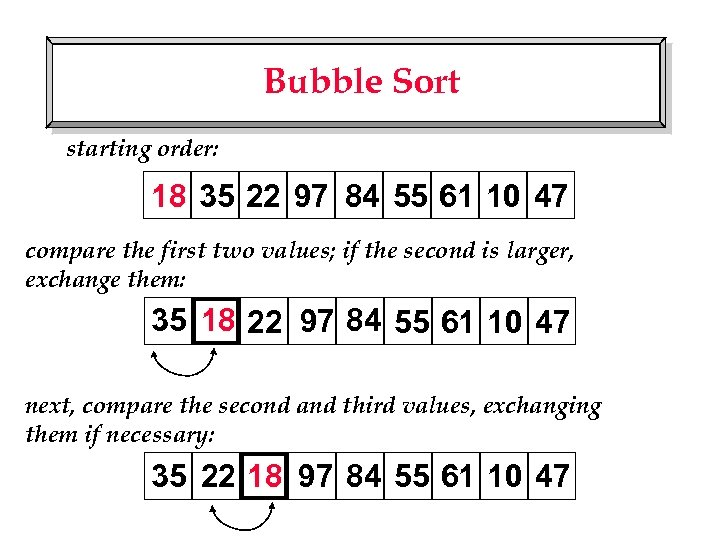Bubble Sort starting order: 18 35 22 97 84 55 61 10 47 compare the first two values; if the second is larger, exchange them: 35 18 22 97 84 55 61 10 47 next, compare the second and third values, exchanging them if necessary: 35 22 18 97 84 55 61 10 47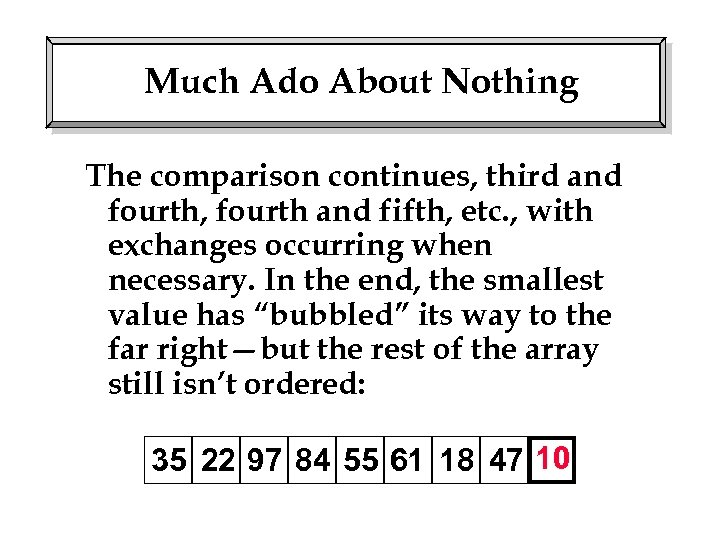Much Ado About Nothing The comparison continues, third and fourth, fourth and fifth, etc. , with exchanges occurring when necessary. In the end, the smallest value has “bubbled” its way to the far right—but the rest of the array still isn’t ordered: 35 22 97 84 55 61 18 47 10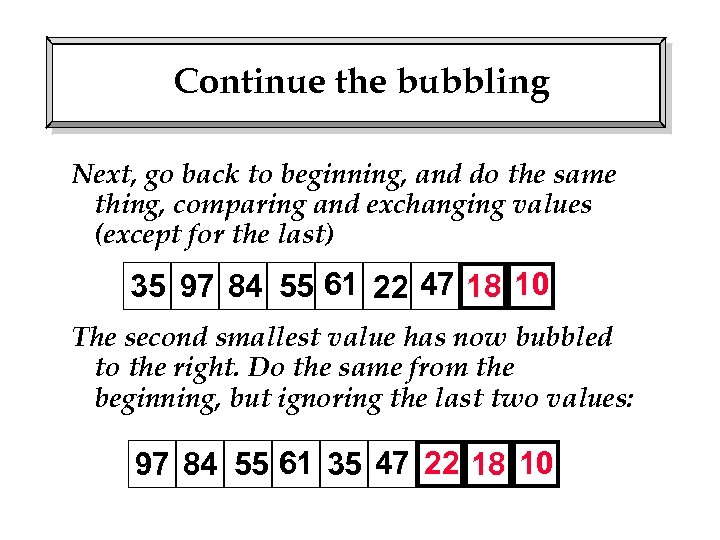Continue the bubbling Next, go back to beginning, and do the same thing, comparing and exchanging values (except for the last) 35 97 84 55 61 22 47 18 10 The second smallest value has now bubbled to the right. Do the same from the beginning, but ignoring the last two values: 97 84 55 61 35 47 22 18 10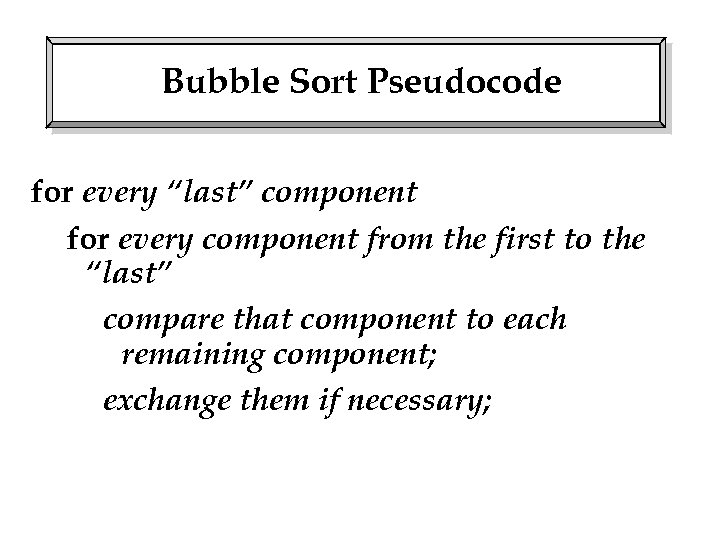Bubble Sort Pseudocode for every “last” component for every component from the first to the “last” compare that component to each remaining component; exchange them if necessary;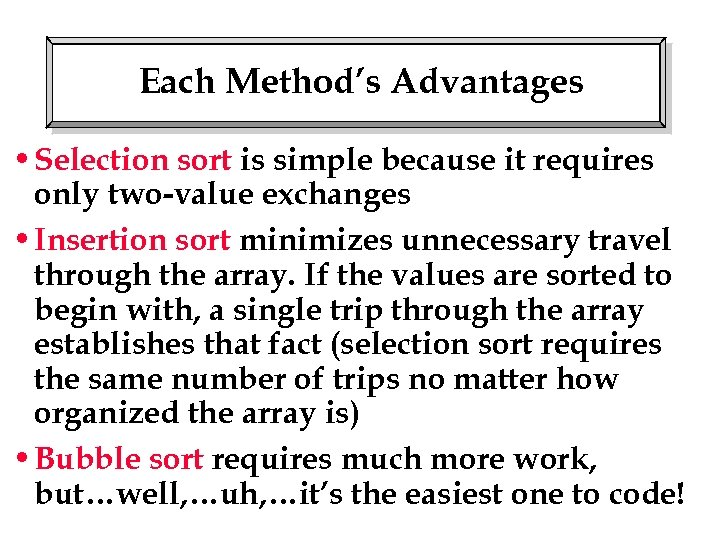Each Method’s Advantages • Selection sort is simple because it requires only two-value exchanges • Insertion sort minimizes unnecessary travel through the array. If the values are sorted to begin with, a single trip through the array establishes that fact (selection sort requires the same number of trips no matter how organized the array is) • Bubble sort requires much more work, but…well, …uh, …it’s the easiest one to code!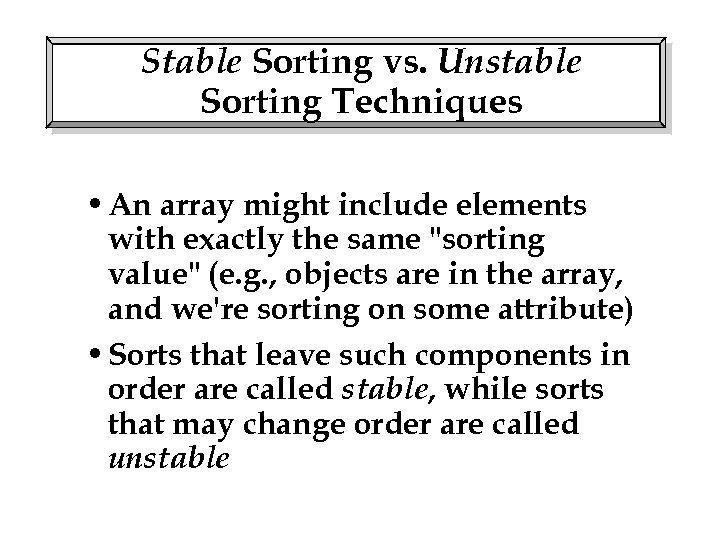Stable Sorting vs. Unstable Sorting Techniques • An array might include elements with exactly the same "sorting value" (e. g. , objects are in the array, and we're sorting on some attribute) • Sorts that leave such components in order are called stable, while sorts that may change order are called unstable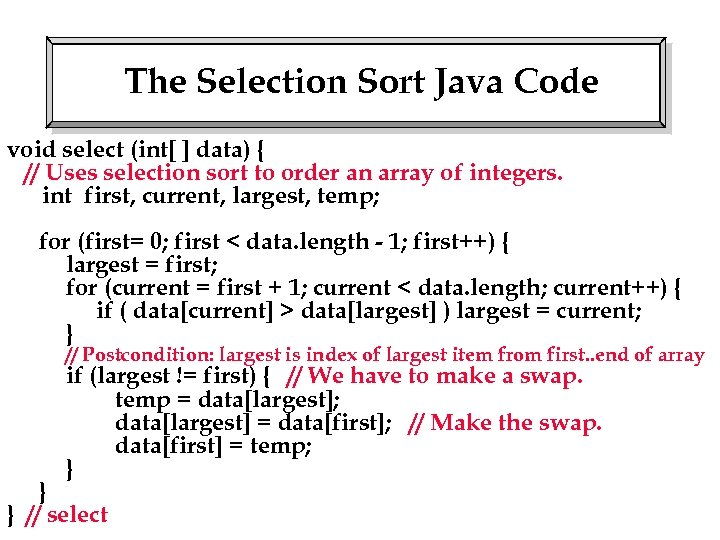The Selection Sort Java Code void select (int[ ] data) { // Uses selection sort to order an array of integers. int first, current, largest, temp; for (first= 0; first < data. length - 1; first++) { largest = first; for (current = first + 1; current < data. length; current++) { if ( data[current] > data[largest] ) largest = current; } // Postcondition: largest is index of largest item from first. . end of array if (largest != first) { // We have to make a swap. temp = data[largest]; data[largest] = data[first]; // Make the swap. data[first] = temp; } } } // select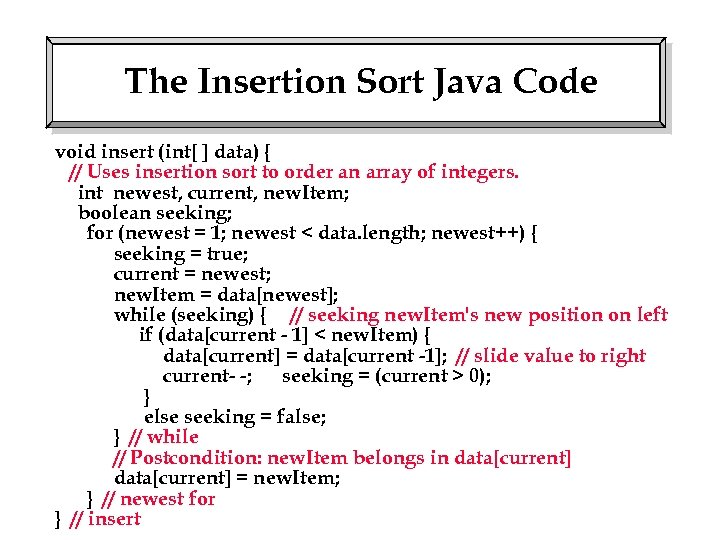The Insertion Sort Java Code void insert (int[ ] data) { // Uses insertion sort to order an array of integers. int newest, current, new. Item; boolean seeking; for (newest = 1; newest < data. length; newest++) { seeking = true; current = newest; new. Item = data[newest]; while (seeking) { // seeking new. Item's new position on left if (data[current - 1] < new. Item) { data[current] = data[current -1]; // slide value to right current- -; seeking = (current > 0); } else seeking = false; } // while // Postcondition: new. Item belongs in data[current] = new. Item; } // newest for } // insert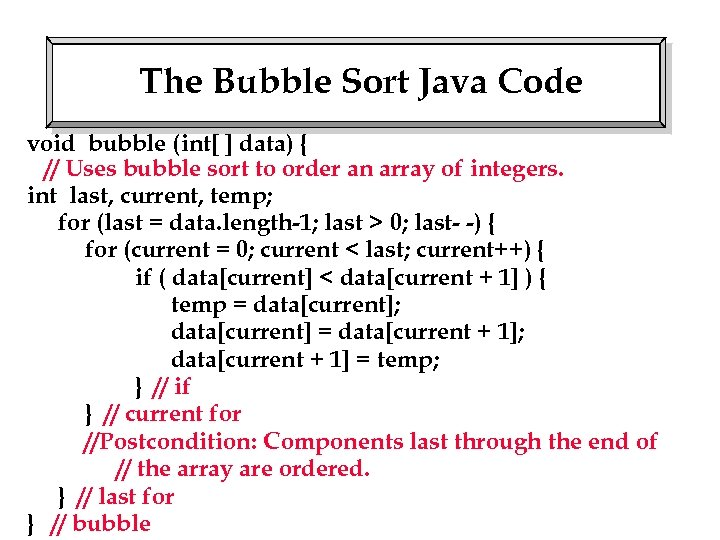The Bubble Sort Java Code void bubble (int[ ] data) { // Uses bubble sort to order an array of integers. int last, current, temp; for (last = data. length-1; last > 0; last- -) { for (current = 0; current < last; current++) { if ( data[current] < data[current + 1] ) { temp = data[current]; data[current] = data[current + 1]; data[current + 1] = temp; } // if } // current for //Postcondition: Components last through the end of // the array are ordered. } // last for } // bubble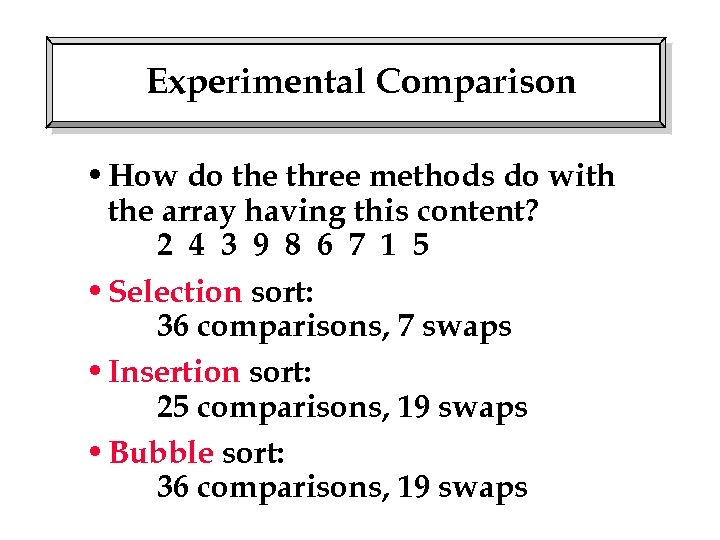Experimental Comparison • How do the three methods do with the array having this content? 2 4 3 9 8 6 7 1 5 • Selection sort: 36 comparisons, 7 swaps • Insertion sort: 25 comparisons, 19 swaps • Bubble sort: 36 comparisons, 19 swaps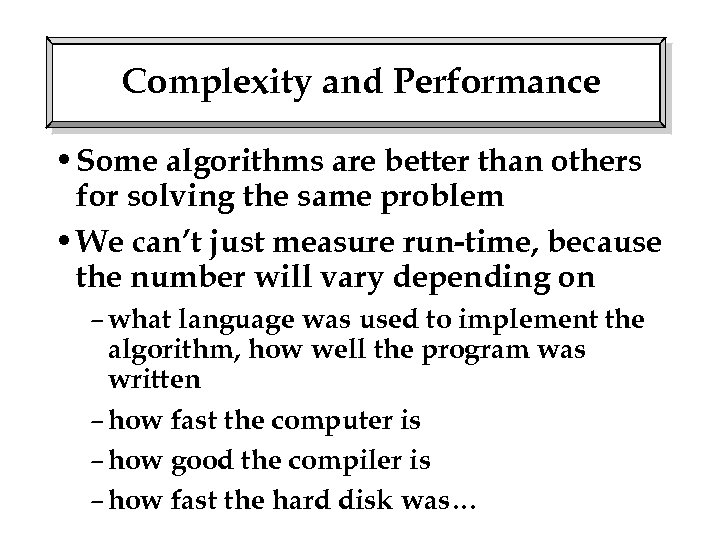Complexity and Performance • Some algorithms are better than others for solving the same problem • We can’t just measure run-time, because the number will vary depending on – what language was used to implement the algorithm, how well the program was written – how fast the computer is – how good the compiler is – how fast the hard disk was…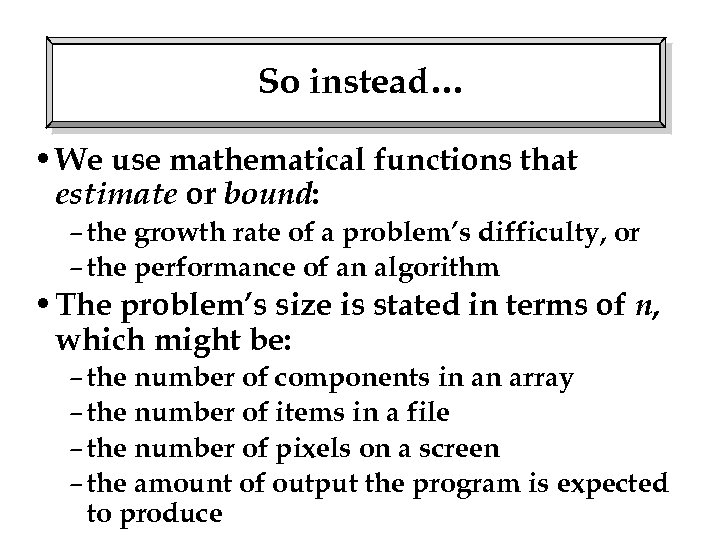So instead… • We use mathematical functions that estimate or bound: – the growth rate of a problem’s difficulty, or – the performance of an algorithm • The problem’s size is stated in terms of n, which might be: – the number of components in an array – the number of items in a file – the number of pixels on a screen – the amount of output the program is expected to produce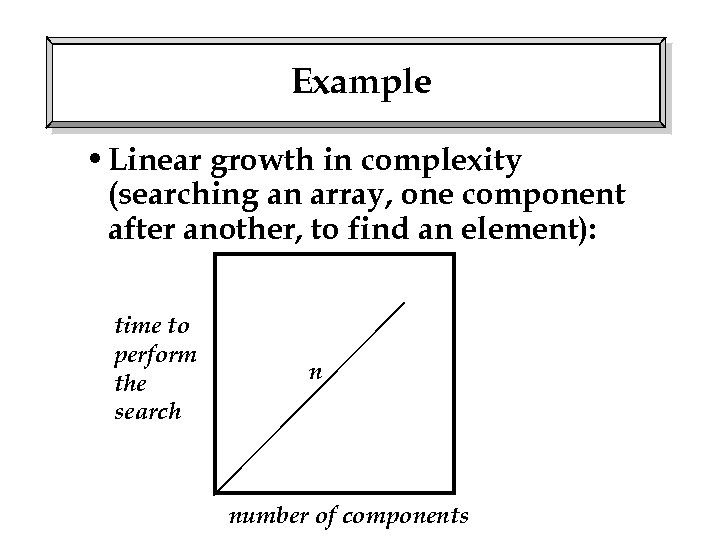Example • Linear growth in complexity (searching an array, one component after another, to find an element): time to perform the search n number of components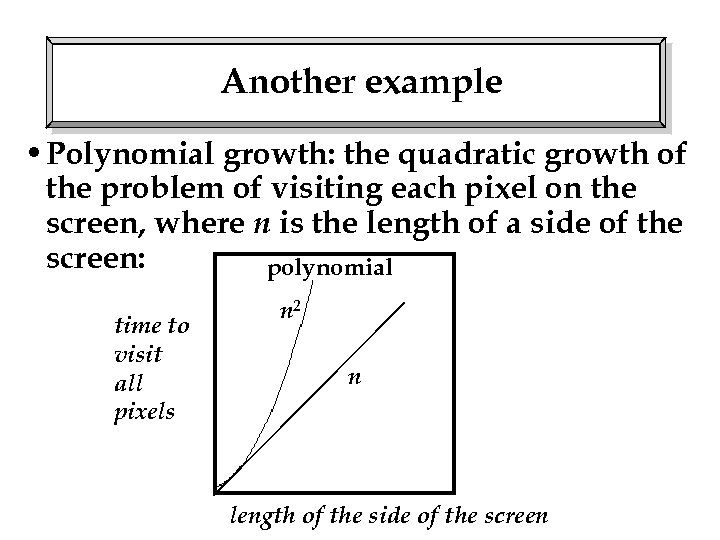Another example • Polynomial growth: the quadratic growth of the problem of visiting each pixel on the screen, where n is the length of a side of the screen: polynomial time to visit all pixels n 2 n length of the side of the screen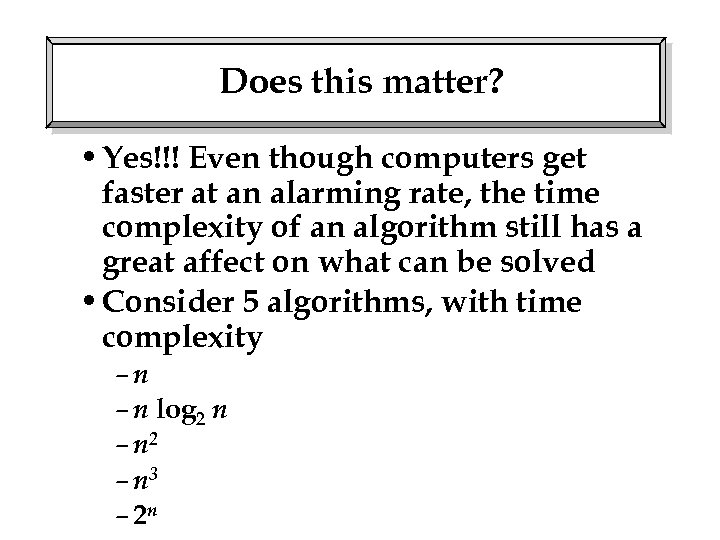Does this matter? • Yes!!! Even though computers get faster at an alarming rate, the time complexity of an algorithm still has a great affect on what can be solved • Consider 5 algorithms, with time complexity –n – n log 2 n – n 2 – n 3 – 2 n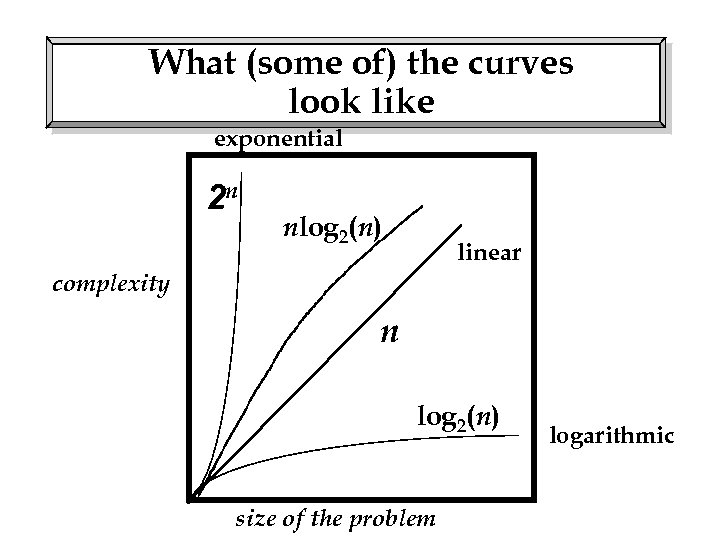What (some of) the curves look like exponential 2 n nlog 2(n) linear complexity n log 2(n) size of the problem logarithmic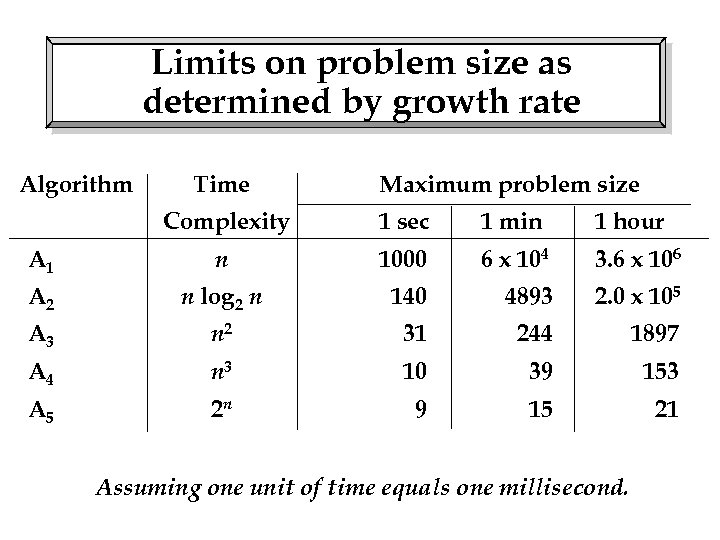Limits on problem size as determined by growth rate Algorithm Time Maximum problem size Complexity 1 sec 1 min 1 hour A 1 n 1000 6 x 104 3. 6 x 106 A 2 n log 2 n 140 4893 2. 0 x 105 A 3 n 2 31 244 1897 A 4 n 3 10 39 153 A 5 2 n 9 15 21 Assuming one unit of time equals one millisecond.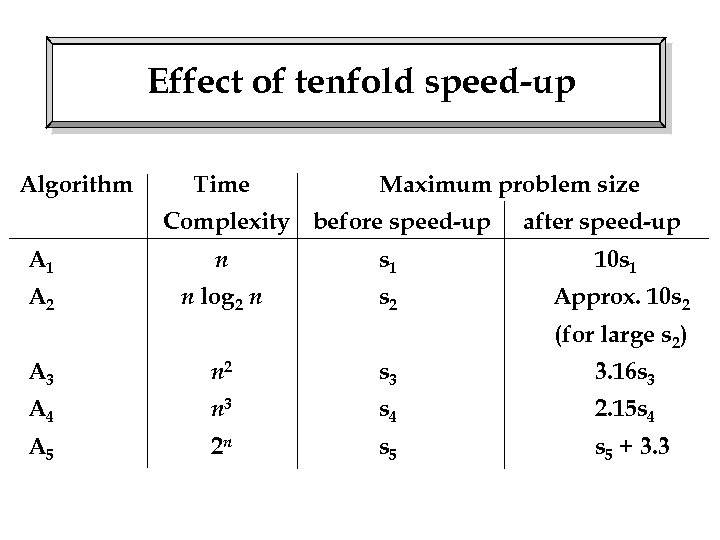Effect of tenfold speed-up Algorithm Time Maximum problem size Complexity before speed-up after speed-up A 1 n s 1 10 s 1 A 2 n log 2 n s 2 Approx. 10 s 2 (for large s 2) A 3 n 2 s 3 3. 16 s 3 A 4 n 3 s 4 2. 15 s 4 A 5 2 n s 5 + 3. 3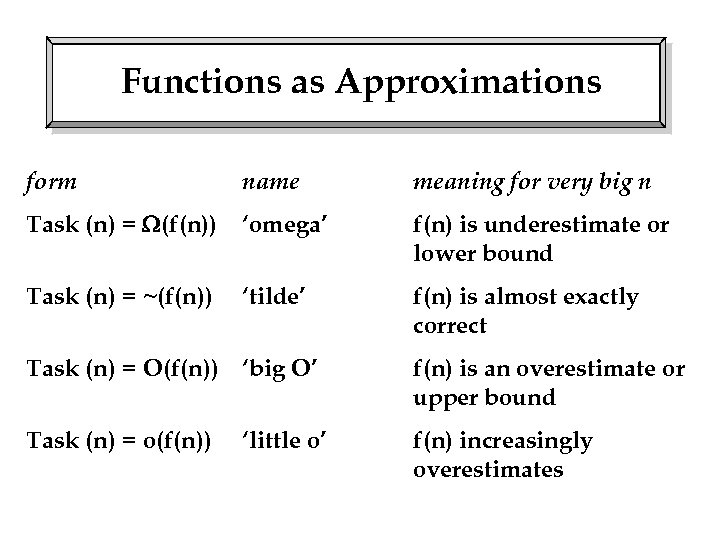Functions as Approximations form name meaning for very big n Task (n) = (f(n)) ‘omega’ f(n) is underestimate or lower bound Task (n) = ~(f(n)) ‘tilde’ f(n) is almost exactly correct Task (n) = O(f(n)) ‘big O’ f(n) is an overestimate or upper bound Task (n) = o(f(n)) f(n) increasingly overestimates ‘little o’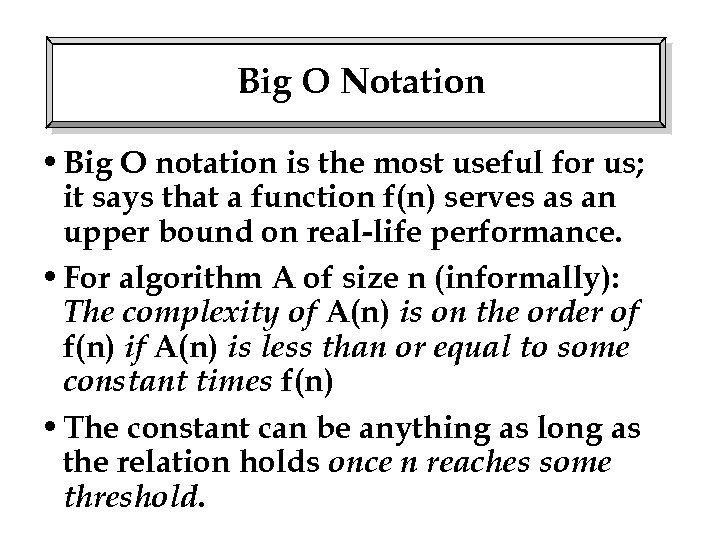Big O Notation • Big O notation is the most useful for us; it says that a function f(n) serves as an upper bound on real-life performance. • For algorithm A of size n (informally): The complexity of A(n) is on the order of f(n) if A(n) is less than or equal to some constant times f(n) • The constant can be anything as long as the relation holds once n reaches some threshold.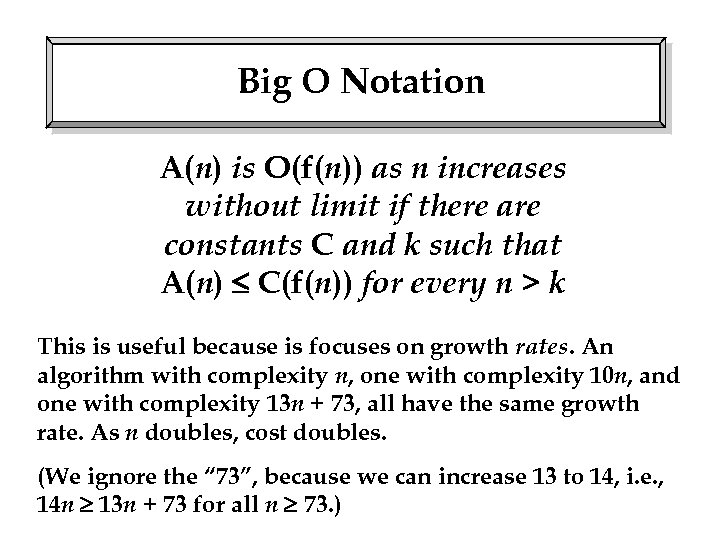Big O Notation A(n) is O(f(n)) as n increases without limit if there are constants C and k such that A(n) C(f(n)) for every n > k This is useful because is focuses on growth rates. An algorithm with complexity n, one with complexity 10 n, and one with complexity 13 n + 73, all have the same growth rate. As n doubles, cost doubles. (We ignore the “ 73”, because we can increase 13 to 14, i. e. , 14 n 13 n + 73 for all n 73. )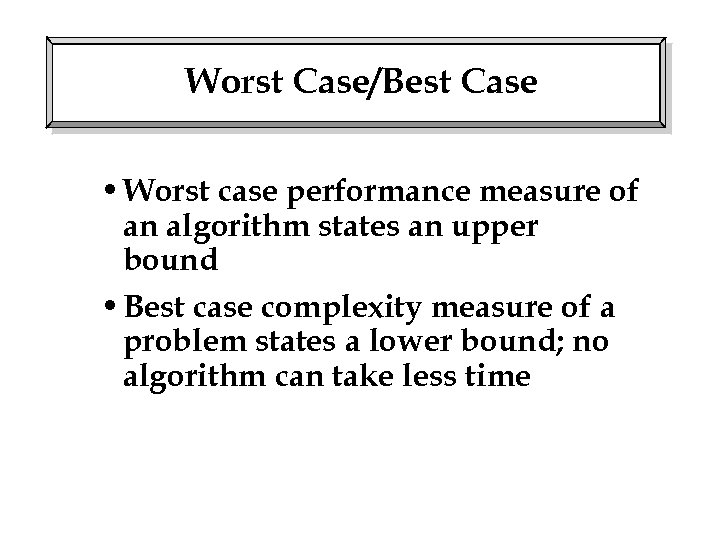Worst Case/Best Case • Worst case performance measure of an algorithm states an upper bound • Best case complexity measure of a problem states a lower bound; no algorithm can take less time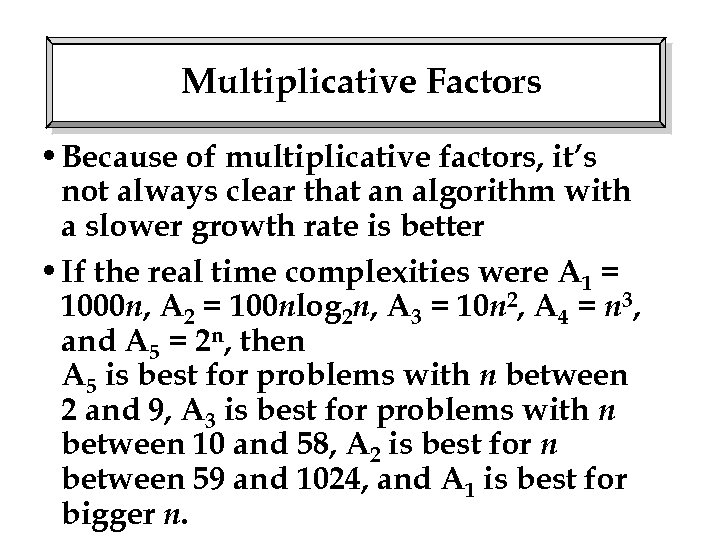Multiplicative Factors • Because of multiplicative factors, it’s not always clear that an algorithm with a slower growth rate is better • If the real time complexities were A 1 = 1000 n, A 2 = 100 nlog 2 n, A 3 = 10 n 2, A 4 = n 3, and A 5 = 2 n, then A 5 is best for problems with n between 2 and 9, A 3 is best for problems with n between 10 and 58, A 2 is best for n between 59 and 1024, and A 1 is best for bigger n.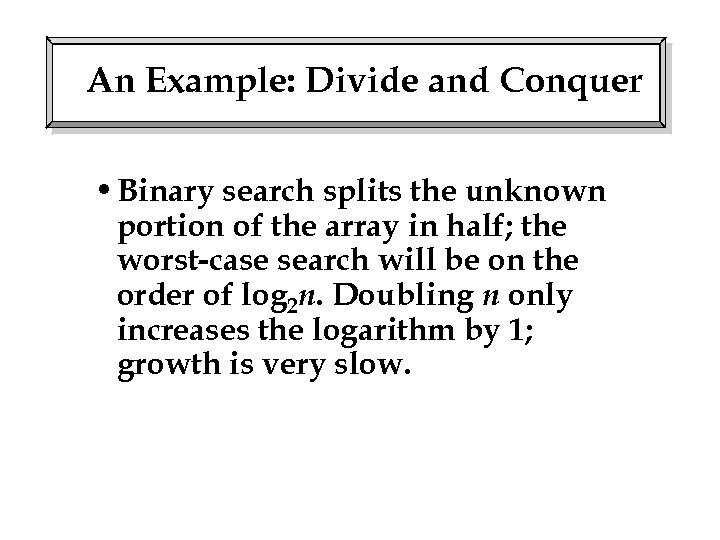An Example: Divide and Conquer • Binary search splits the unknown portion of the array in half; the worst-case search will be on the order of log 2 n. Doubling n only increases the logarithm by 1; growth is very slow.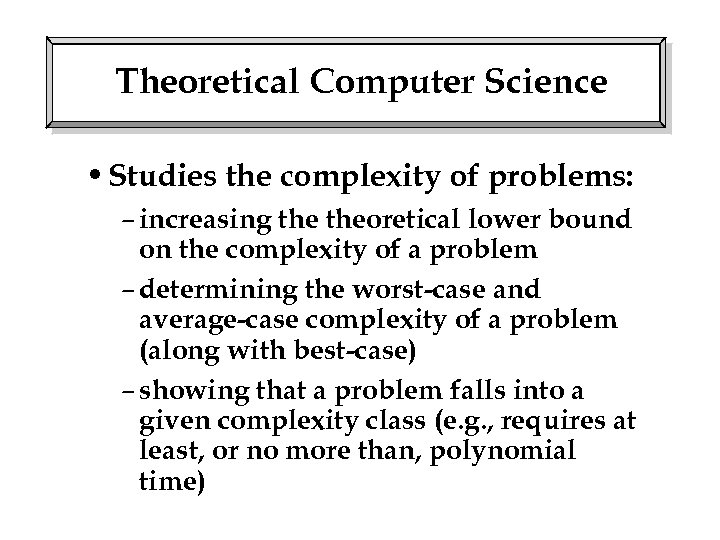Theoretical Computer Science • Studies the complexity of problems: – increasing theoretical lower bound on the complexity of a problem – determining the worst-case and average-case complexity of a problem (along with best-case) – showing that a problem falls into a given complexity class (e. g. , requires at least, or no more than, polynomial time)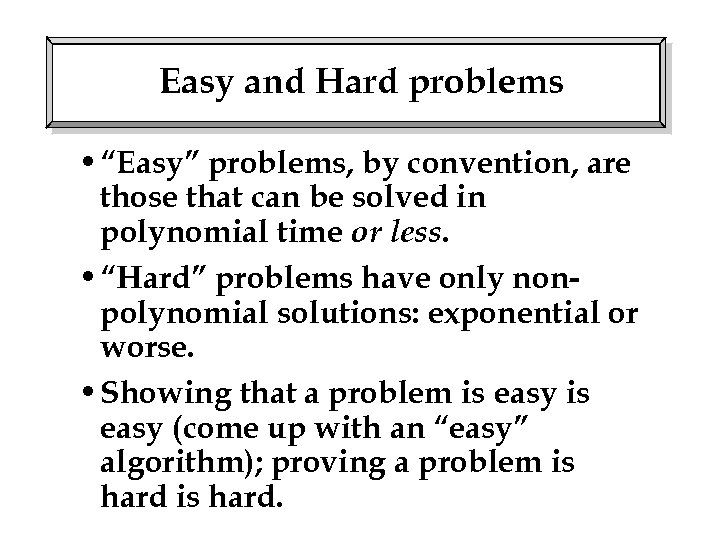Easy and Hard problems • “Easy” problems, by convention, are those that can be solved in polynomial time or less. • “Hard” problems have only nonpolynomial solutions: exponential or worse. • Showing that a problem is easy (come up with an “easy” algorithm); proving a problem is hard.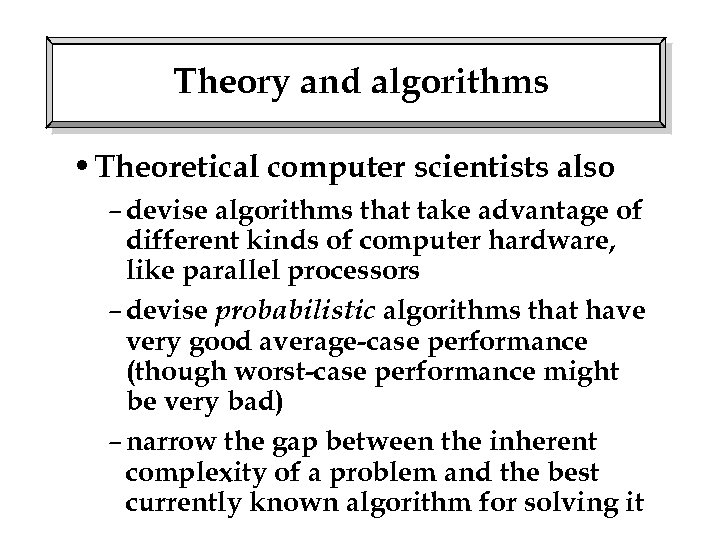Theory and algorithms • Theoretical computer scientists also – devise algorithms that take advantage of different kinds of computer hardware, like parallel processors – devise probabilistic algorithms that have very good average-case performance (though worst-case performance might be very bad) – narrow the gap between the inherent complexity of a problem and the best currently known algorithm for solving it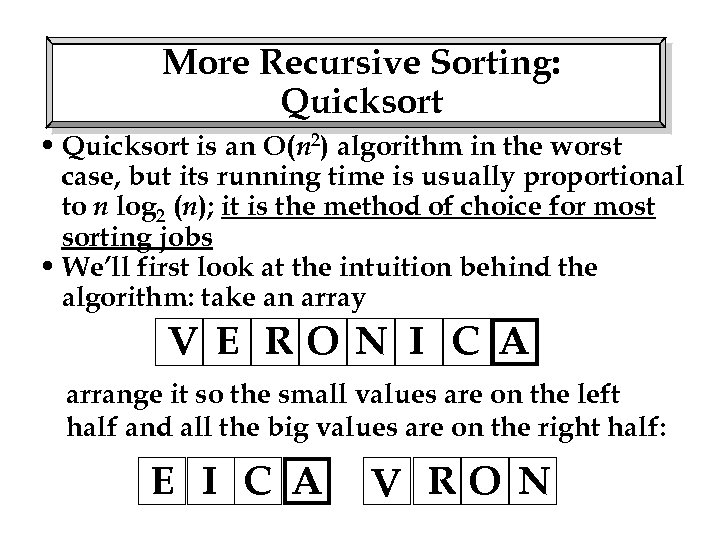More Recursive Sorting: Quicksort • Quicksort is an O(n 2) algorithm in the worst case, but its running time is usually proportional to n log 2 (n); it is the method of choice for most sorting jobs • We’ll first look at the intuition behind the algorithm: take an array V E RO N I C A arrange it so the small values are on the left half and all the big values are on the right half: E I C A V RO N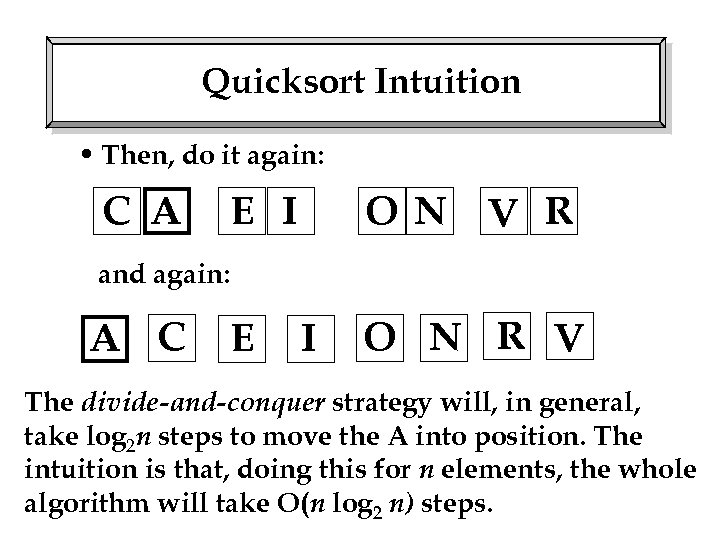Quicksort Intuition • Then, do it again: C A E I O N V R and again: A C E I O N R V The divide-and-conquer strategy will, in general, take log 2 n steps to move the A into position. The intuition is that, doing this for n elements, the whole algorithm will take O(n log 2 n) steps.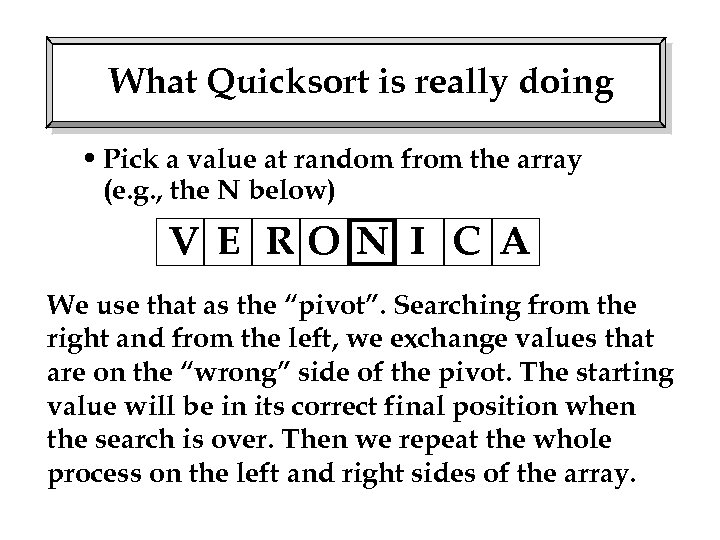What Quicksort is really doing • Pick a value at random from the array (e. g. , the N below) V E RO N I C A We use that as the “pivot”. Searching from the right and from the left, we exchange values that are on the “wrong” side of the pivot. The starting value will be in its correct final position when the search is over. Then we repeat the whole process on the left and right sides of the array.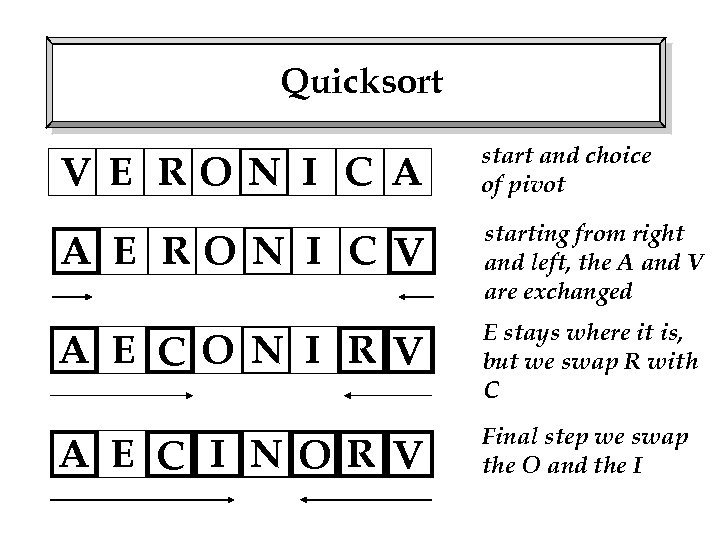Quicksort V E RO N I C A start and choice of pivot A E RO N I C V starting from right and left, the A and V are exchanged A E CO N I R V E stays where it is, but we swap R with C A E C I NO R V Final step we swap the O and the I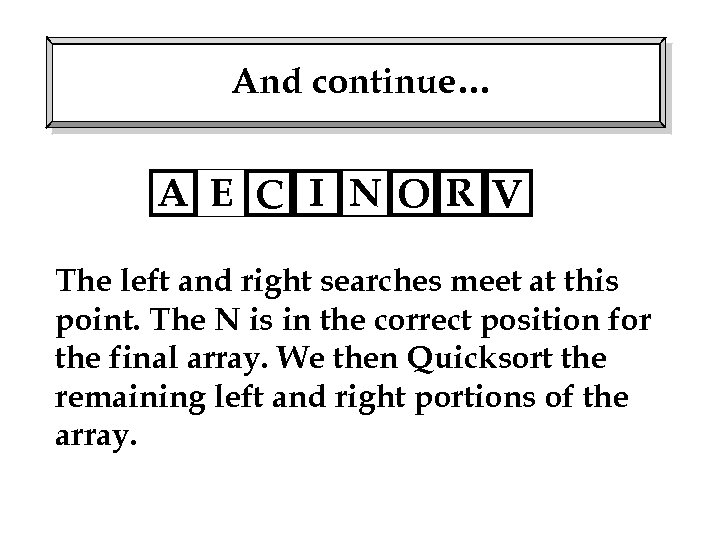And continue… A E C I NO R V The left and right searches meet at this point. The N is in the correct position for the final array. We then Quicksort the remaining left and right portions of the array.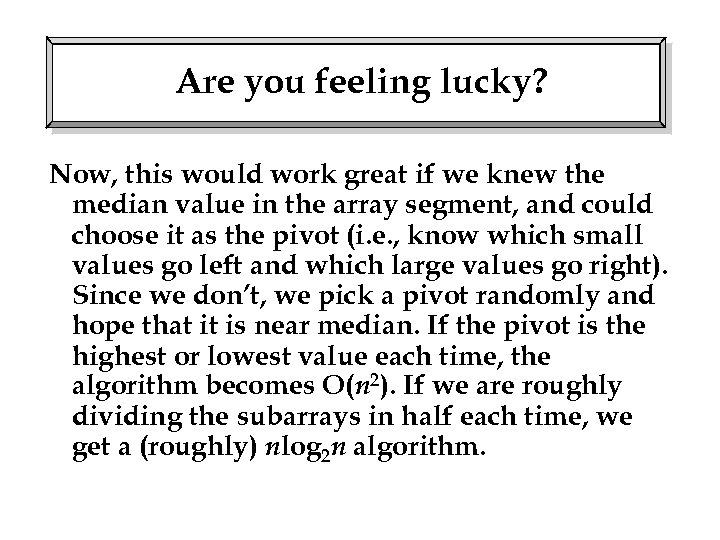Are you feeling lucky? Now, this would work great if we knew the median value in the array segment, and could choose it as the pivot (i. e. , know which small values go left and which large values go right). Since we don’t, we pick a pivot randomly and hope that it is near median. If the pivot is the highest or lowest value each time, the algorithm becomes O(n 2). If we are roughly dividing the subarrays in half each time, we get a (roughly) nlog 2 n algorithm.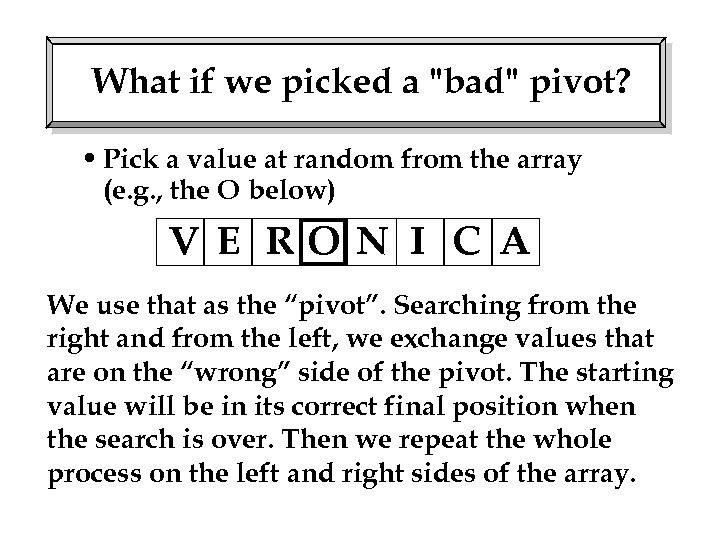What if we picked a "bad" pivot? • Pick a value at random from the array (e. g. , the O below) V E RO N I C A We use that as the “pivot”. Searching from the right and from the left, we exchange values that are on the “wrong” side of the pivot. The starting value will be in its correct final position when the search is over. Then we repeat the whole process on the left and right sides of the array.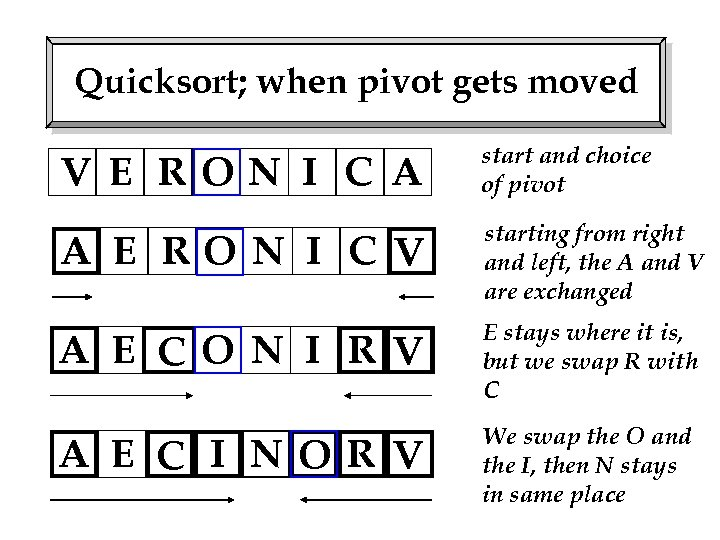Quicksort; when pivot gets moved V E RON I C A start and choice of pivot A E RO N I C V starting from right and left, the A and V are exchanged A E CO N I R V E stays where it is, but we swap R with C A E C I NO R V We swap the O and the I, then N stays in same place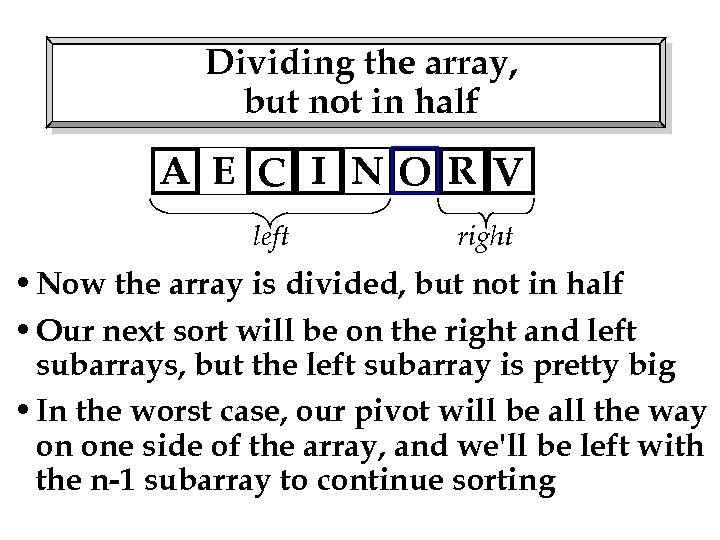Dividing the array, but not in half A E C I NO R V left right • Now the array is divided, but not in half • Our next sort will be on the right and left subarrays, but the left subarray is pretty big • In the worst case, our pivot will be all the way on one side of the array, and we'll be left with the n-1 subarray to continue sorting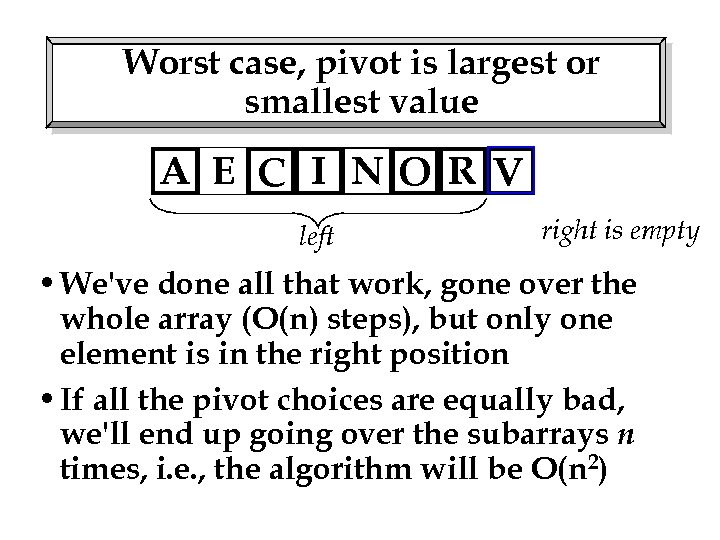Worst case, pivot is largest or smallest value A E C I NO R V left right is empty • We've done all that work, gone over the whole array (O(n) steps), but only one element is in the right position • If all the pivot choices are equally bad, we'll end up going over the subarrays n times, i. e. , the algorithm will be O(n 2)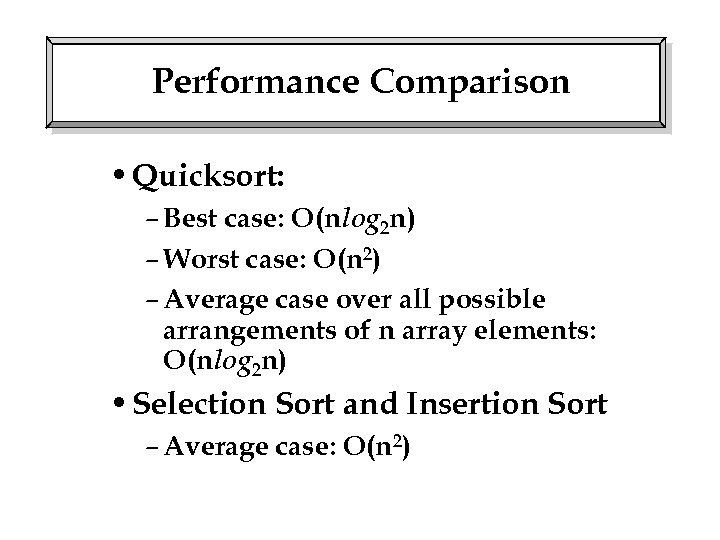Performance Comparison • Quicksort: – Best case: O(nlog 2 n) – Worst case: O(n 2) – Average case over all possible arrangements of n array elements: O(nlog 2 n) • Selection Sort and Insertion Sort – Average case: O(n 2)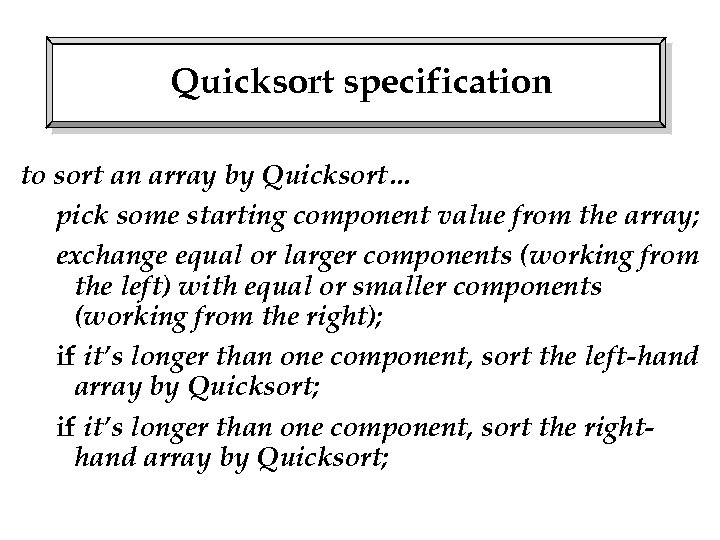Quicksort specification to sort an array by Quicksort… pick some starting component value from the array; exchange equal or larger components (working from the left) with equal or smaller components (working from the right); if it’s longer than one component, sort the left-hand array by Quicksort; if it’s longer than one component, sort the righthand array by Quicksort;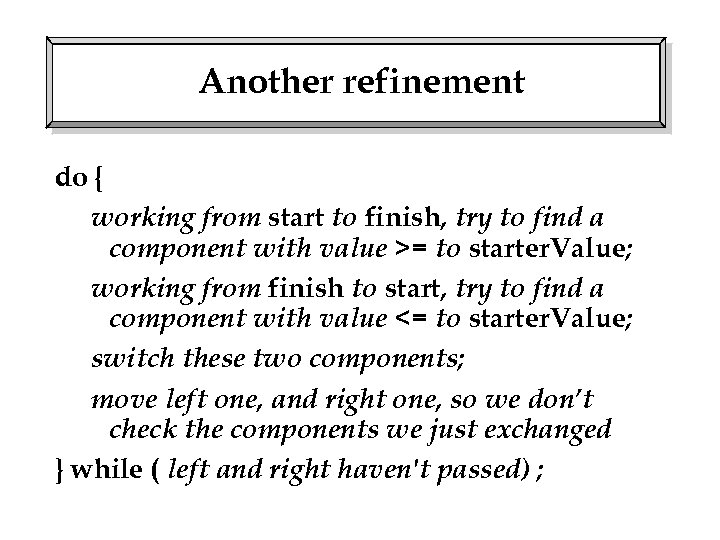Another refinement do { working from start to finish, try to find a component with value >= to starter. Value; working from finish to start, try to find a component with value <= to starter. Value; switch these two components; move left one, and right one, so we don’t check the components we just exchanged } while ( left and right haven't passed) ;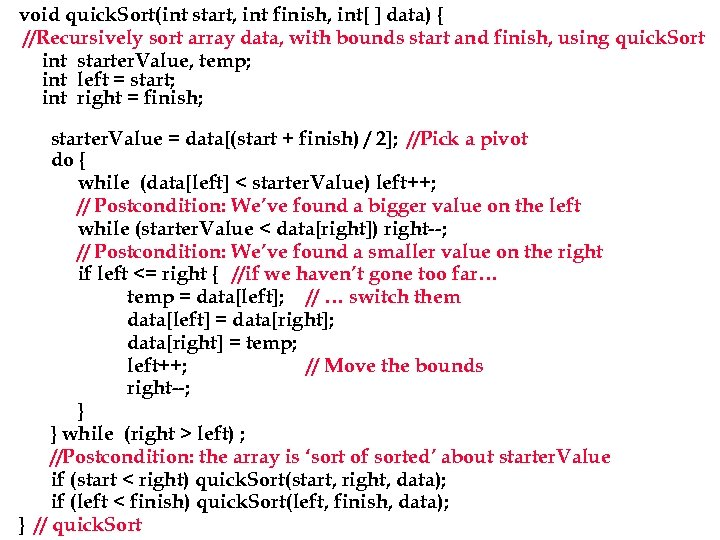void quick. Sort(int start, int finish, int[ ] data) { //Recursively sort array data, with bounds start and finish, using quick. Sort int starter. Value, temp; int left = start; int right = finish; starter. Value = data[(start + finish) / 2]; //Pick a pivot do { while (data[left] < starter. Value) left++; // Postcondition: We’ve found a bigger value on the left while (starter. Value < data[right]) right--; // Postcondition: We’ve found a smaller value on the right if left <= right { //if we haven’t gone too far… temp = data[left]; // … switch them data[left] = data[right]; data[right] = temp; left++; // Move the bounds right--; } } while (right > left) ; //Postcondition: the array is ‘sort of sorted’ about starter. Value if (start < right) quick. Sort(start, right, data); if (left < finish) quick. Sort(left, finish, data); } // quick. Sort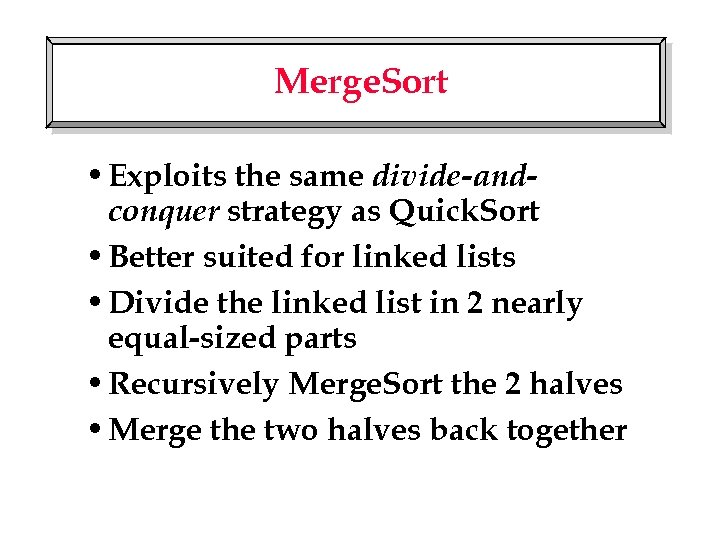Merge. Sort • Exploits the same divide-andconquer strategy as Quick. Sort • Better suited for linked lists • Divide the linked list in 2 nearly equal-sized parts • Recursively Merge. Sort the 2 halves • Merge the two halves back together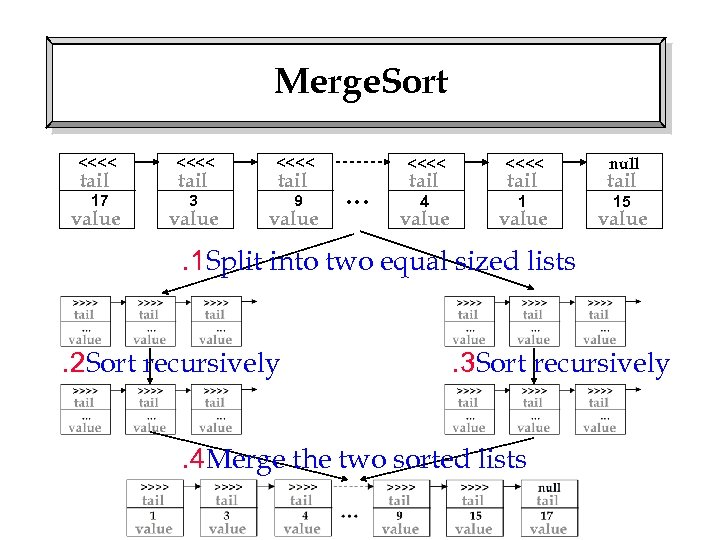Merge. Sort <<<< 17 3 9 tail value <<<< … <<<< 4 1 tail value null tail value 15 . 1 Split into two equal sized lists. 2 Sort recursively . 3 Sort recursively . 4 Merge the two sorted listsWhat Makes Up Merge. Sort? • We need to be able to carry out two operations • Splitting a linked list into two pieces • Merging two ordered linked lists into a single ordered linked list • Let's look at merging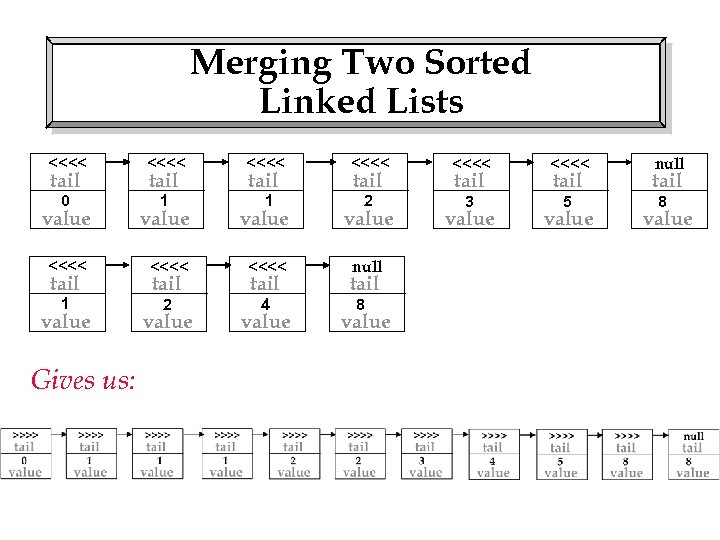Merging Two Sorted Linked Lists <<<< <<<< 0 1 1 2 3 5 tail value <<<< null 1 2 4 tail value Gives us: tail value 8 tail value null tail value 8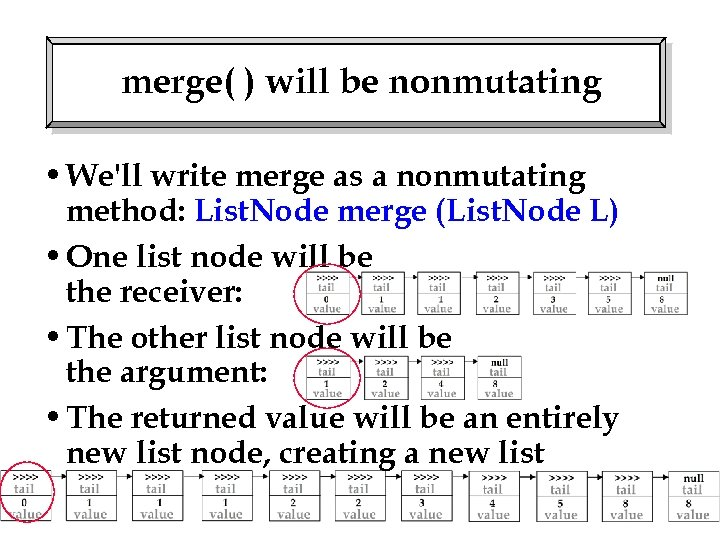merge( ) will be nonmutating • We'll write merge as a nonmutating method: List. Node merge (List. Node L) • One list node will be the receiver: • The other list node will be the argument: • The returned value will be an entirely new list node, creating a new list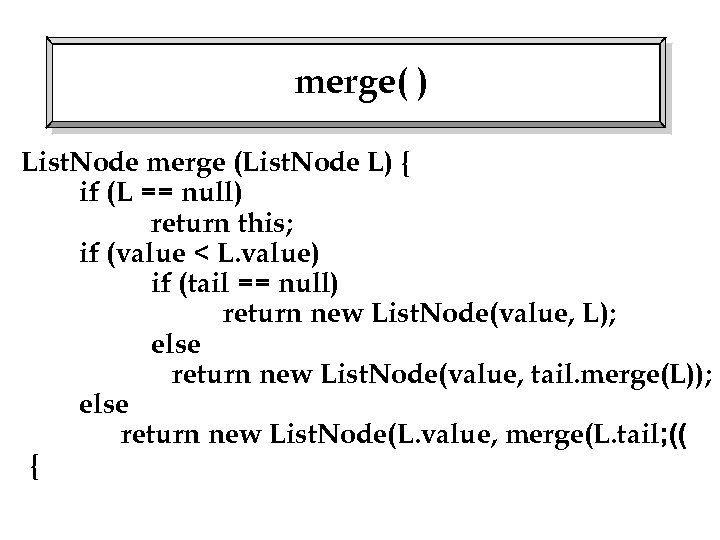merge( ) List. Node merge (List. Node L) { if (L == null) return this; if (value < L. value) if (tail == null) return new List. Node(value, L); else return new List. Node(value, tail. merge(L)); else return new List. Node(L. value, merge(L. tail; (( {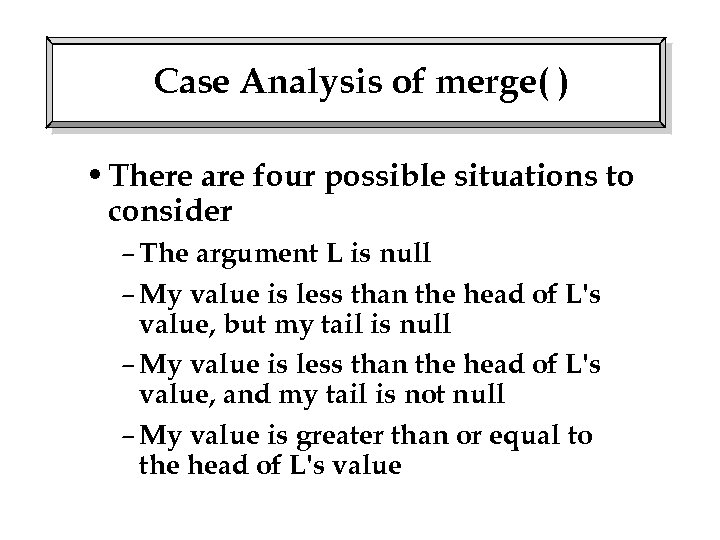Case Analysis of merge( ) • There are four possible situations to consider – The argument L is null – My value is less than the head of L's value, but my tail is null – My value is less than the head of L's value, and my tail is not null – My value is greater than or equal to the head of L's value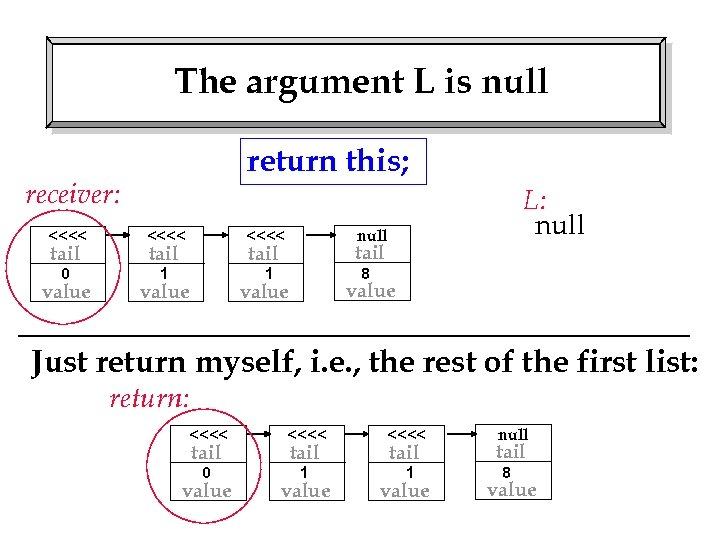The argument L is null return this; receiver: <<<< 0 1 null <<<< 1 tail value L: null tail value 8 Just return myself, i. e. , the rest of the first list: return: <<<< 0 1 1 tail value null tail value 8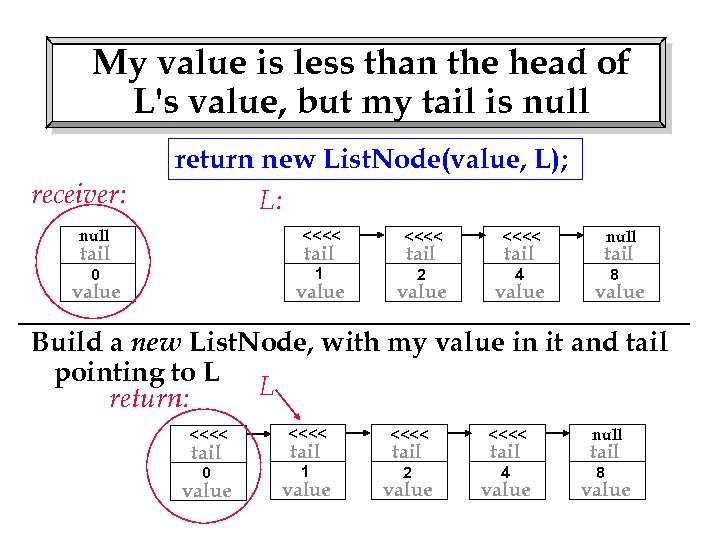My value is less than the head of L's value, but my tail is null receiver: return new List. Node(value, L); L: null <<<< 0 1 2 4 tail value null tail value 8 Build a new List. Node, with my value in it and tail pointing to L L return: <<<< 0 1 2 4 tail value null tail value 8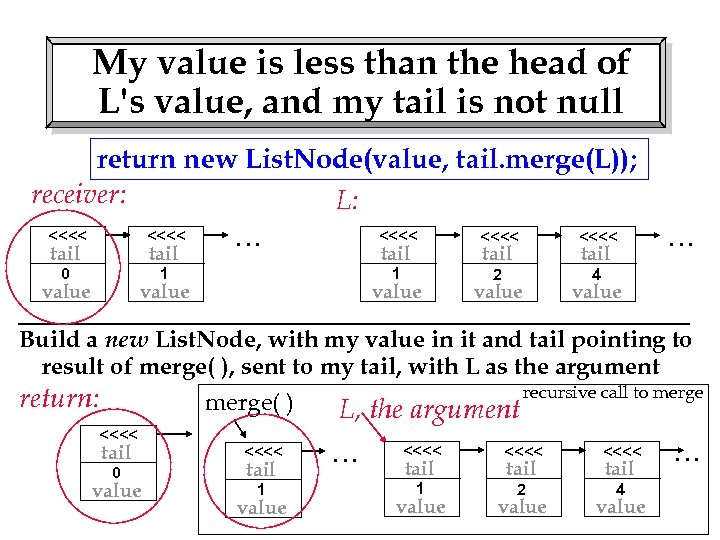My value is less than the head of L's value, and my tail is not null return new List. Node(value, tail. merge(L)); receiver: L: <<<< 0 … 1 tail value <<<< 1 2 4 tail value … value tail value Build a new List. Node, with my value in it and tail pointing to result of merge( ), sent to my tail, with L as the argument return: merge( ) <<<< tail <<<< value 1 0 tail value L, the argument … recursive call to merge <<<< 1 2 4 tail value …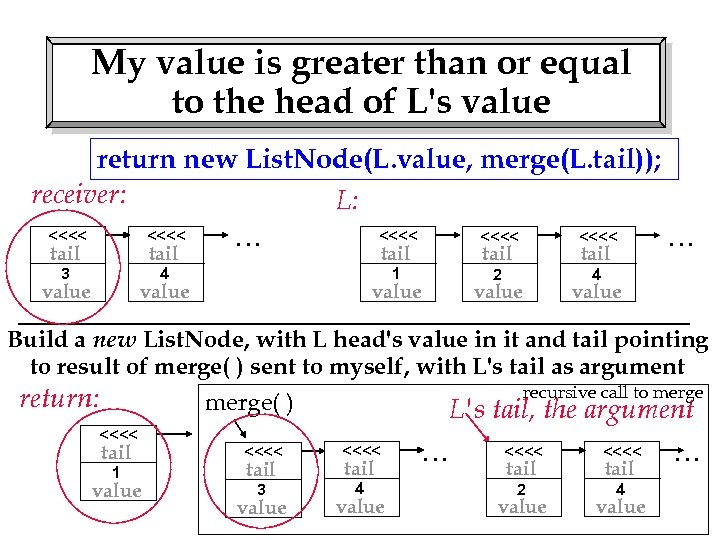My value is greater than or equal to the head of L's value return new List. Node(L. value, merge(L. tail)); receiver: L: <<<< 3 … 4 tail value <<<< 1 2 4 tail value … tail value Build a new List. Node, with L head's value in it and tail pointing to result of merge( ) sent to myself, with L's tail as argument return: recursive call to merge( ) <<<< L's tail, the argument tail <<<< value 3 4 1 tail value … <<<< 2 4 tail value …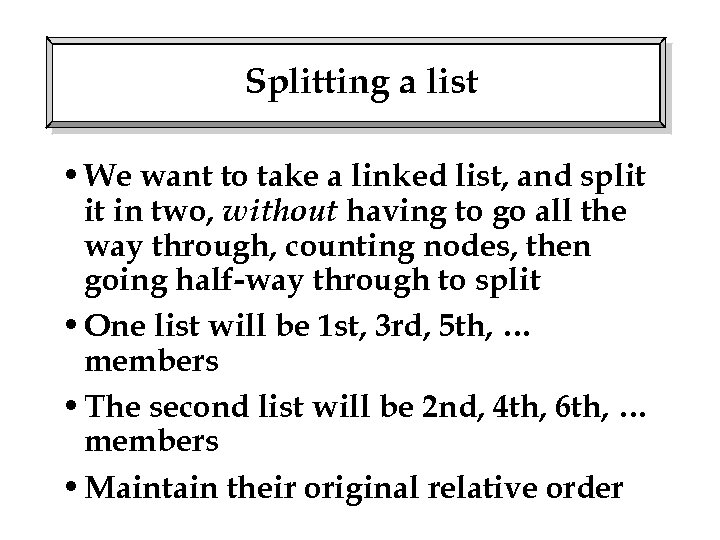Splitting a list • We want to take a linked list, and split it in two, without having to go all the way through, counting nodes, then going half-way through to split • One list will be 1 st, 3 rd, 5 th, … members • The second list will be 2 nd, 4 th, 6 th, … members • Maintain their original relative order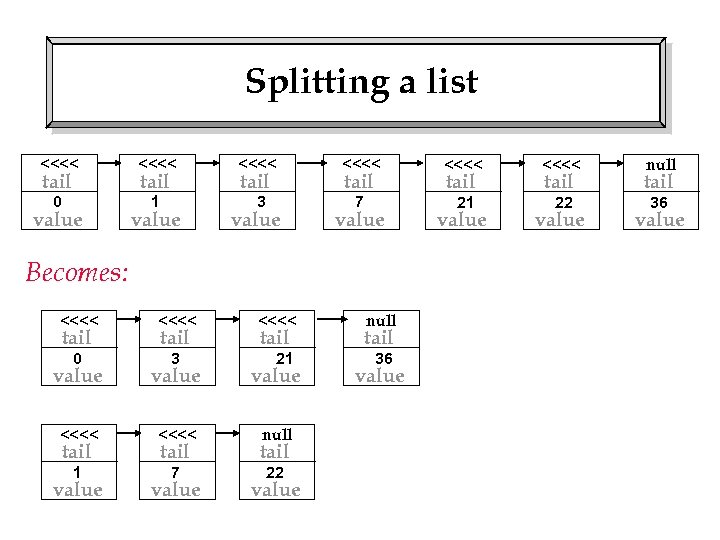Splitting a list <<<< <<<< 0 1 3 7 21 22 tail value Becomes: <<<< 0 3 tail <<<< tail value 21 value <<<< null 1 7 tail value null tail value 22 36 tail value null tail value 36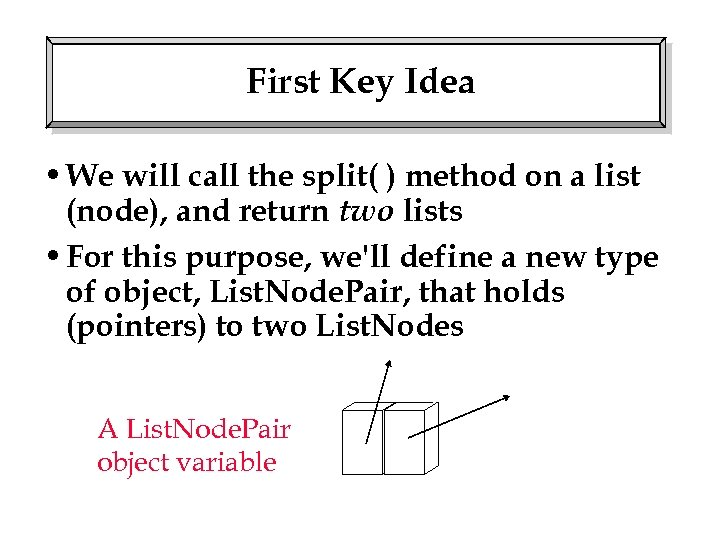First Key Idea • We will call the split( ) method on a list (node), and return two lists • For this purpose, we'll define a new type of object, List. Node. Pair, that holds (pointers) to two List. Nodes A List. Node. Pair object variable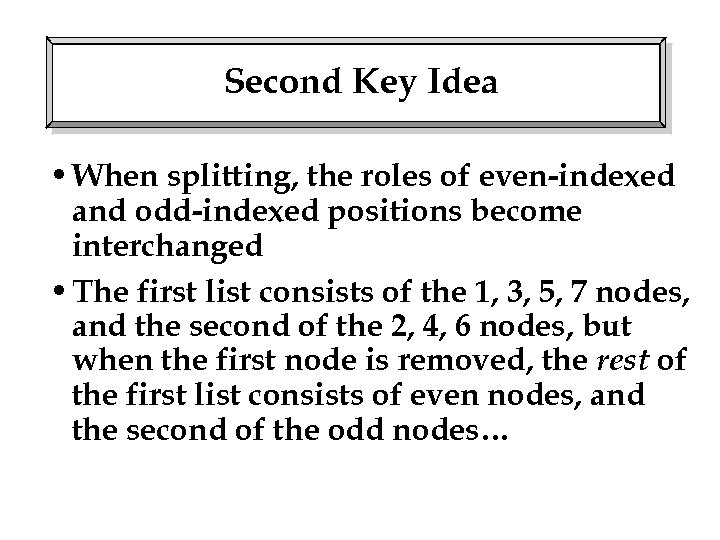Second Key Idea • When splitting, the roles of even-indexed and odd-indexed positions become interchanged • The first list consists of the 1, 3, 5, 7 nodes, and the second of the 2, 4, 6 nodes, but when the first node is removed, the rest of the first list consists of even nodes, and the second of the odd nodes…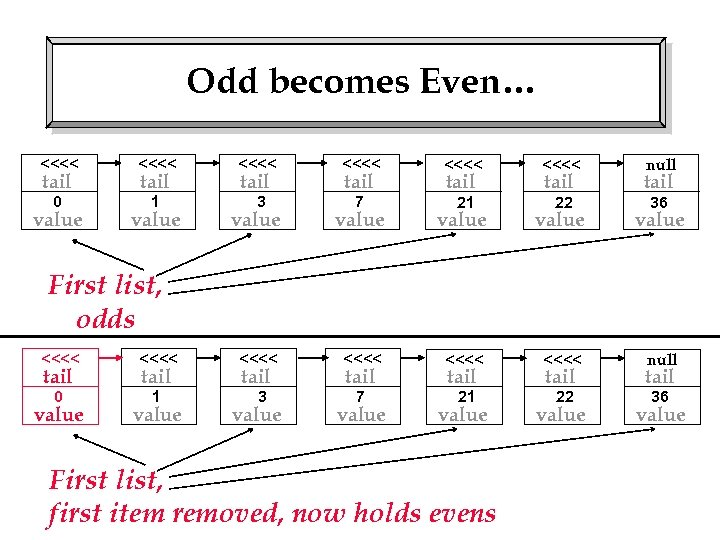Odd becomes Even… <<<< <<<< 0 1 3 7 21 22 tail value tail null tail 36 value value null First list, odds <<<< <<<< 0 1 3 7 21 22 tail value tail value First list, first item removed, now holds evens tail value 36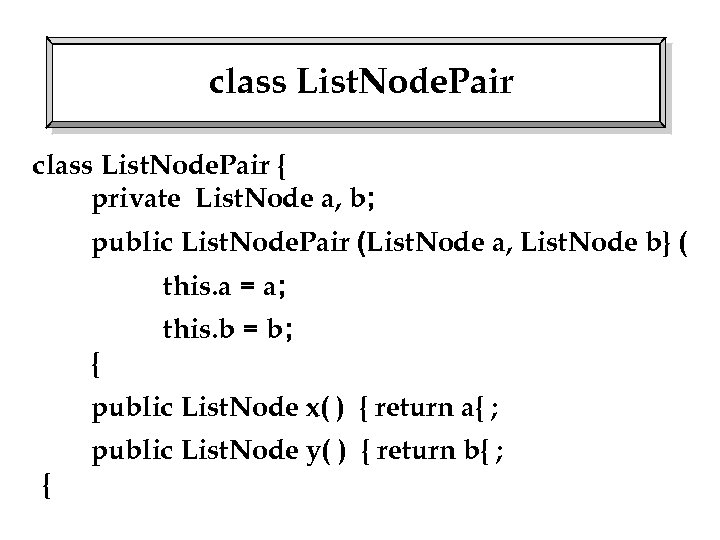class List. Node. Pair { private List. Node a, b; public List. Node. Pair (List. Node a, List. Node b} ( this. a = a; this. b = b; { public List. Node x( ) { return a{ ; public List. Node y( ) { return b{ ; {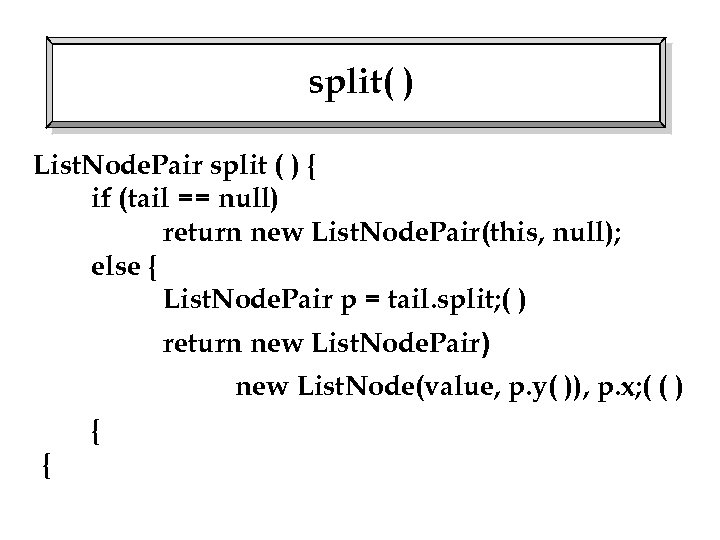split( ) List. Node. Pair split ( ) { if (tail == null) return new List. Node. Pair(this, null); else { List. Node. Pair p = tail. split; ( ) return new List. Node. Pair) new List. Node(value, p. y( )), p. x; ( ( ) { {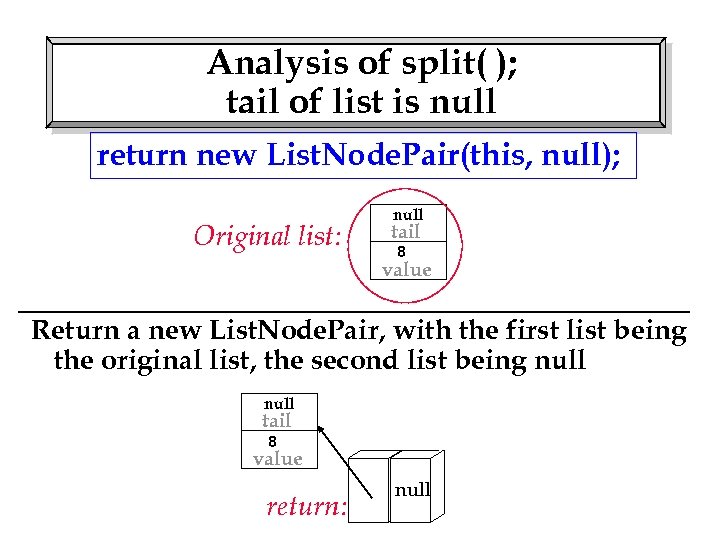Analysis of split( ); tail of list is null return new List. Node. Pair(this, null); Original list: null tail 8 value Return a new List. Node. Pair, with the first list being the original list, the second list being null tail 8 value return: null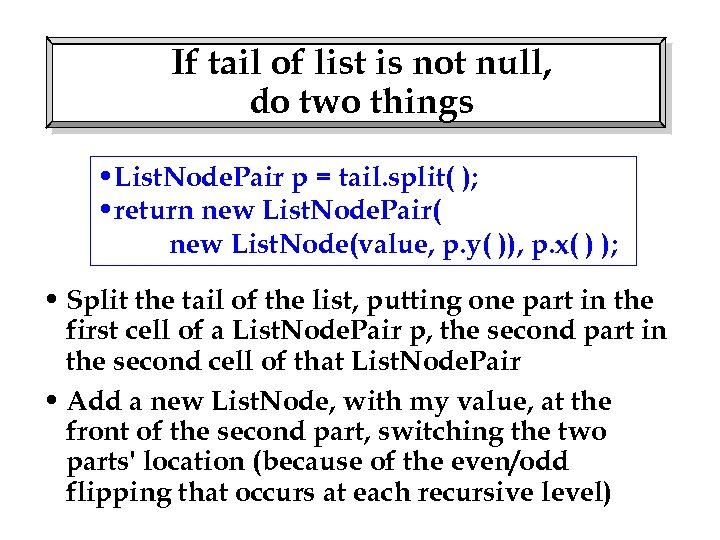If tail of list is not null, do two things • List. Node. Pair p = tail. split( ); • return new List. Node. Pair( new List. Node(value, p. y( )), p. x( ) ); • Split the tail of the list, putting one part in the first cell of a List. Node. Pair p, the second part in the second cell of that List. Node. Pair • Add a new List. Node, with my value, at the front of the second part, switching the two parts' location (because of the even/odd flipping that occurs at each recursive level)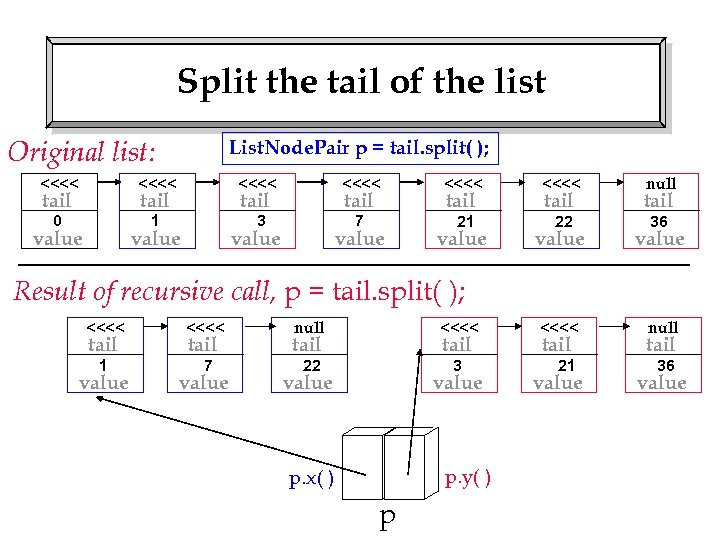Split the tail of the list Original list: List. Node. Pair p = tail. split( ); <<<< <<<< 0 1 3 7 21 22 tail value tail value null tail value <<<< null 36 Result of recursive call, p = tail. split( ); <<<< 1 7 tail value null <<<< 22 3 tail value p. y( ) p. x( ) p tail value 21 36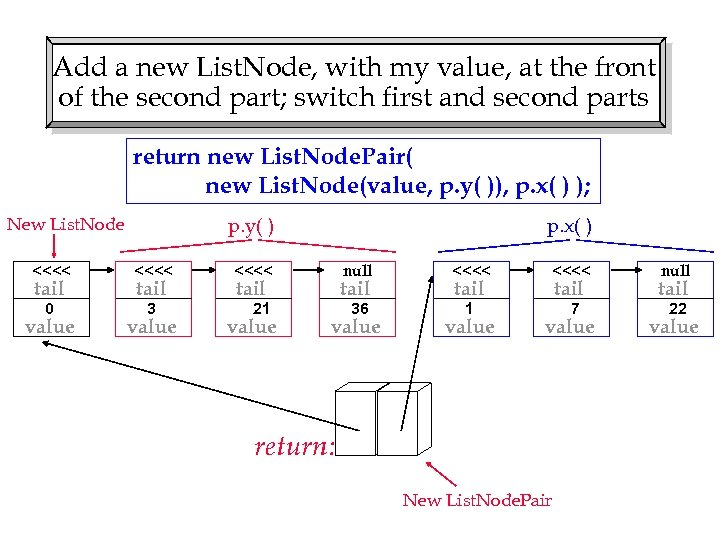Add a new List. Node, with my value, at the front of the second part; switch first and second parts return new List. Node. Pair( new List. Node(value, p. y( )), p. x( ) ); p. y( ) New List. Node <<<< 0 3 tail value p. x( ) <<<< null <<<< 36 1 7 tail value 21 tail value null tail value return: New List. Node. Pair 22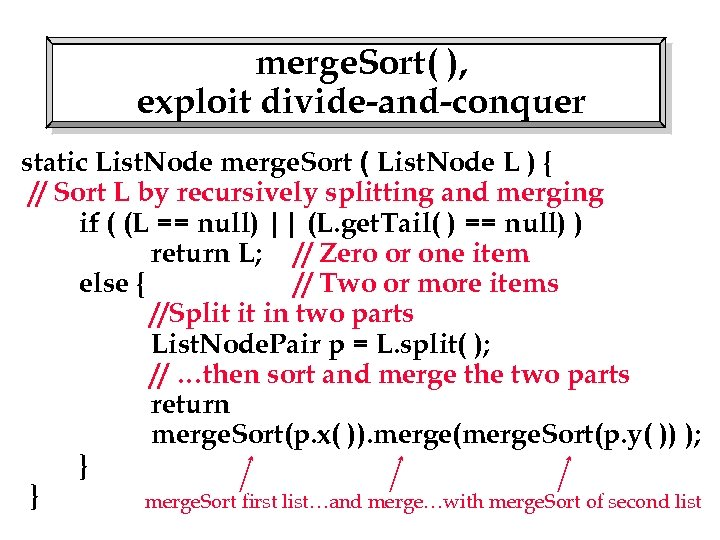merge. Sort( ), exploit divide-and-conquer static List. Node merge. Sort ( List. Node L ) { // Sort L by recursively splitting and merging if ( (L == null) || (L. get. Tail( ) == null) ) return L; // Zero or one item else { // Two or more items //Split it in two parts List. Node. Pair p = L. split( ); // …then sort and merge the two parts return merge. Sort(p. x( )). merge(merge. Sort(p. y( )) ); } } merge. Sort first list…and merge…with merge. Sort of second list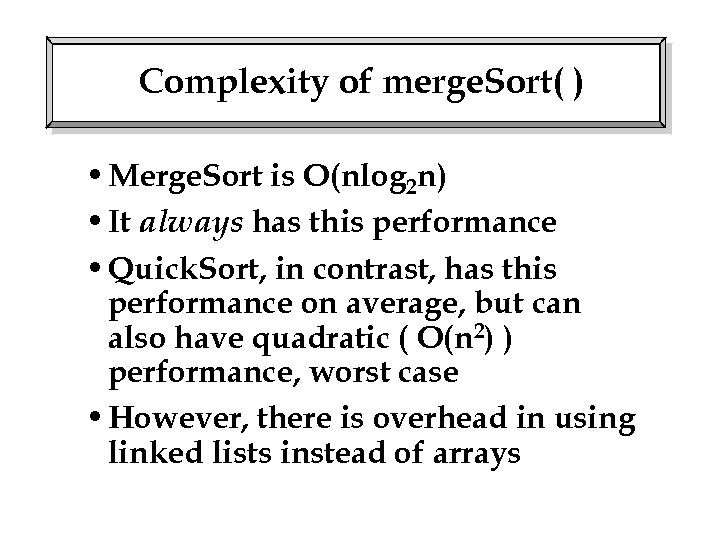Complexity of merge. Sort( ) • Merge. Sort is O(nlog 2 n) • It always has this performance • Quick. Sort, in contrast, has this performance on average, but can also have quadratic ( O(n 2) ) performance, worst case • However, there is overhead in using linked lists instead of arrays